CONTENTS 32pg 171K 32fig 1. Introduction 2. Modern Energy Science 3. Defining energy 4. Theories of what fills the universe 5. Reasons for a Theory of Everything 6. Space continuum, Force fields, Wavicles, Theory of Everything 7. Observer and absolute systems 8. Space continuum, Force fields, Wavicles 9. Wavicles vs particles and waves 10. External energy vs internal energy 11. First and second laws of energy 12. Second-law evaluations 13. Forms of energy 14. Units 15. Temperature of internal energy 16. Types of mass and energy 17. Momentum, impulse, acceleration, forces 18. Energy vs work and heat 19. Caloric energy 20. Helmholtz energy 21. The carnot ratio and helmholtz ratio 22. Helmholtz ratio vs entropy 23. Reversible and irreversible processes 24. Energy transformation 25. Efficiency terms 26. Is perpetual motion possible? 27. Hydro-power 28. Solar energy conversion 29. Gas turbine 30. Electrochemical fuel cells 31. Refrigerator 32. Food calories to muscle power 33. Energy science history 34. Symbols 35. Notes 36. References 37. Revision history >>>Copyright 2008Aug11 by Ben Wiens...energy scientist 1. INTRODUCTION     Understanding how energy behaves especially on the more microscopic theoretical level is not easy for even those with university science degrees. Present advanced concepts with names such as thermodynamics, entropy, relativity, quantum mechanics, quantum field theory, and string theories are difficult to comprehend. Often complicated formulas and scientific jargon are used.     In this booklet all the concepts of energy are presented in a reasonably uncomplicated way. While these concepts presented are founded on modern accepted scientific information, many of the explanations are different than in present popular books. Concise definitions are provided that can be used in the same way in all disciplines of science and result in a quicker understanding of the concepts presented. Understanding energy through concepts rather than extensive formulas is stressed.     The focus of this booklet is to provide concepts of energy that can be useful in general education, for general interest, as well as being useful in practical applications in the fields of engineering, physics, chemistry and biology. Rating Year 12 minimum.     It is a good idea to open the Encyclopedia of Energy Science in a new window and use it as a glossary for this booklet.Fig 1 Not many people understand present energy concepts such as entropy 2. MODERN ENERGY SCIENCE     Traditionally Engineering, Physics, Chemistry and Biology all focus on concepts of energy in a different way. For example more complex energy concepts in Engineering are often limited to topics involving thermodynamics. This branch of science adopts the older Joule or classical view of energy. In Physics the much bigger and modern Einstein picture of energy based on Relativity and Quantum theories is popular. In Chemistry the study of energy is often restricted to a small subset of thermodynamic topics mixed with quantum theories. In Biology the study of energy is severely restricted to a small subset of Chemistry energy topics.     Many modern scientific and engineering developments involve energy concepts from many scientific disciplines. Modern scientists and engineers therefore should be more familiar with energy concepts outside of their specific areas. It is beneficial then to group the basic concepts of energy from the different areas of science together. Such a common study of energy is called energy science.     Our current understanding of energy comes from different time periods, different disciplines of science, and different theories or models. When studying energy science one should be aware of which model is being used. Sometimes an old model is used because it is simpler to understand but this does not result in a knowledge of the latest ideas on the topic. It would be better to find a simple way of explaining a current model. Sometimes a convenient model is used for ease of understanding only but is not necessarily labeled as such. Other times there are competing models based on different theories. At times these competing models are even bundled together. Other times complex terms are not defined. Definitions are important because words can often represent many paragraphs of thinking. If energy science is taught by mixing up current, old, convenient and competing models along with definitions that are not spelled out, then a lot of confusion can result.     In this booklet the current view is always used but this is not always based on the most popular explanation, rather it is an attempt at reviewing the information and weaving a single best thread through the current competing models available. 3. DEFINING ENERGY?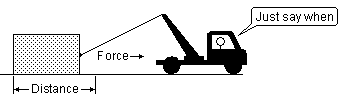Fig 2 Energy is the ability to create a force over a distance some time in the future     Energy is not that old a word, Thomas Young proposed it in 1807 . Initially energy was thought of as the ability to do macroscopic work like the work the kinetic energy of a cannonball can do on impact or the work that the potential energy of a hoisted block of granite can do on falling. Work was defined as...a force acting over a distance. Later in 1830 Joule said that this energy or ability to do macroscopic work was also contained within the molecules. He suggested that the molecules contained forms of microscopic energy just like that contained in the moving cannonball and the hoisted block of granite. In 1905 Einstein suggested that matter such as the molecules and atoms are really very large collections of energy particles. As well there existed energy particles such as the photon that transferred energy between different types of matter. Under some conditions some of these energy particles could be liberated from the atoms in different forms such as light and heat. When we feel the weight of an apple in our hand we are really feeling the weight of the energy particles in the apple. How could energy be defined then according to modern science ? Energy...the ability to create a force over a distance in the universe from the very smallest event within the tiniest of particles to the very largest between celestial objects in the universe. Work...a force acting over a distance in the universe from the very smallest event within the tiniest of particles to the very largest between celestial objects in the universe. Energy then is the ability to do work some time in the future. It is stored work potential. Work is the actual act of the force acting over a distance.     The definition of energy however is a rather small part of what energy science is all about. First of all energy such as matter and photons is not the only "entity" that appears to exist in the universe. There is a space continuum and there are force fields that are not considered to be made of energy or matter. It is the properties of the entire system and how energy behaves in this system that are of most interest in energy science. 4. THEORIES OF WHAT FILLS THE UNIVERSE     Many theories have been proposed over time as to what fills up the universe. Even the Greeks had their theories. Aristotle concluded that everything on the earth was made of four substances; earth, water, air and fire. The universe outside of the earth was made of a fifth substance called aether. In 1633 Rene Descartes proposed that the universe was entirely full of three elements. All material things were made of the third element. The second element's particles made up a fluid called the aether that filled the space in-between the third element. The first element was what the aether particles were made of. Light was not made of the third element but was rather a vibration that traveled in the aether fluid. In the theory proposed by Isaac Newton around 1676, light as well as all physical objects were made of particles that travel in empty space. He got most scientists to accept this idea during the 1700s. Newton based his theory on his experiments with prisms. Many phenomena of light could not be explained if light was a wave such as a sound wave. In 1801 Thomas Young produced an interference pattern with light and pronounced light was a wave traveling in a material aether, so most scientists again changed their views. Scientists believed the aether not only provided a medium for light waves to travel in but also did the job of controlling the speed of light just as the properties of air control the speed of sound traveling through it. Physical objects however were considered to be particles.     Fitzeau's tests in 1849 showed that the speed of light was extremely constant. It was theorized that the aether was stationary at some point in the universe. If this was true, it was likely that the earth moved through this stationary aether. In 1887 the famous Michelson-Morley test, which used two beams of light traveling perpendicular to each other, failed to detect the motion of the earth through the aether. This startled many scientists and they began looking for other theories that would explain the behavior of light. Lorentz felt that the instruments Michelson and Morley had used may have changed in length in the direction of travel relative to the aether. Poincare a leading French mathematician felt that our time system may be altered as we travel through the aether.     Theories about how mass and energy behaved as they traveled through the universe were being developed long before Einstein stated his famous theories. Even the formulae E=m·c2 it appears was put on paper in 1888 by J.J. Thomson. With Einstein came the turning point in beginning to fathom the much bigger picture of how the universe behaved. In 1905 Einstein wrote two separate theories regarding the behavior of energy in the universe. The first is now labeled as the Quantum theory and described how energy is emitted and absorbed on the microscopic level as particles or quanta of energy as opposed to waves such as sound waves. The second is called the Special Relativity theory and it described how light and matter changed energy as they traveled relative to space-time. In 1916 Einstein published the General Relativity theory which described a more complex relationship between space, mass, energy, motion and gravity. He proposed that energy did not travel in a material aether as had been thought. The properties of space-time, he suggested, were considerably different. Einstein also worked on a Unified field theory, where he hoped to reveal how all forces were really made up of a common entity, but never succeeded.     In the 1920s Quantum Mechanics became popular. Some variations of this theory viewed particles as wavelike while others viewed all microscopic particles as points with probabilistic outcome of their behavior. It does not take into account the relativistic factors in high speed particles. In 1986 the string theory and a little later the superstring theory , Membrane theory, and around 1998 the M-theory  were proposed which claimed that all matter and force particles are the result of different nodes of vibrating strings or membranes. Other scientists claim that strings are just ideas at this point and there is not definite proof, we should be thinking more in terms of a broader more open discussion forum where a wide range of possibilities can be discussed. This broader view has recently been labeled the Theory of Everything.

 Symbol Description LET Lorentz aether theory...the latest aether theory before special relativity SR Einstein Special Relativity theory...all motion is relative in the universe QT Einstein Quantum Theory...light is emitted as wavelike particles GR Einstein General Relativity theory...gravity causes space to be curved QM Quantum Mechanics...all energy is composed of particles and probabilities of location UFT Unified Field Theory...attempt at finding common entity for all four forces QFT Quantum Field Theory...forces are the result of different types of force particles GUT Grand Unified Theory...unification of strong, weak, electromagnetic interactions TUT Totally Unified Theory...explain working of universe down to single particle TOE Theory of Everything...try to explain working of the universe as the biggest picture

 Fig 3 Major theories of how the universe behaves 5. REASONS FOR A THEORY OF EVERYTHING     It is a well known fact that Einstein's Special Relativity and General Relativity are not complete theories of the universe. They explain nothing of how the microscopic world of energy relates. Einstein is also father of the Quantum theory which describes how energy is exchanged on the microscopic level as vibrating particles. It is interesting however that even Einstein, who developed both the Relativity and Quantum theories, did not ever combine the two together. Each of the theories explains a part of how the universe operates by using models that are valid only in a very limited sense. These theories can be described as partial theories. Quantum Mechanics is also a partial theory. Presently we do have modern models or theories that are much more comprehensive so these theories should be talked about only in a historical sense.     It is not likely that we will know exactly how the universe operates to the smallest detail for many years. Perhaps the smallest entity of space will be too small for us to ever measure and identify. Even though we will not know about the biggest picture for some time, it is best that a modern theory such as the Theory of Everything be used. Only the framework of such a system is presently understood. It is better to use such a modern view as opposed to hanging on to theories such as Relativity and Quantum mechanics which hold partial and opposing concepts of the universe. The Theory of Everything therefore will not be a complete picture presently but it will be filled in slowly over time. It is at least an attempt at painting the biggest picture. If we see a large canvas we know that there is much left to fill in. If we have a small canvas which is nearly full, we think that there is little left to paint in. The Theory of Everything can be used to encompass all the different theories as long as they propose to describe the biggest picture possible.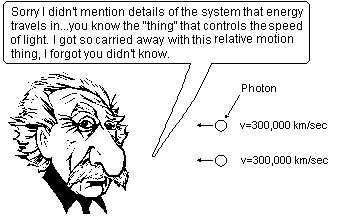Fig 4 Einstein rarely mentioned details of the space continuum that energy travels in     One of the major things that is missing from most of the theories is, what fills space. For example Einstein's Special Relativity theory renounced the aether as the medium that light traveled in. Many people to this day think that Einstein's Special Relativity theory proposed that light and matter traveled in space that was totally void. Though it was not mentioned in the theory, Einstein later cleared up this matter by saying that "this rigid four-dimensional space of the Special theory of Relativity is to some extent a four-dimensional analogue of H.A. Lorentz's rigid three-dimensional aether" . So Einstein's space-time in his Special Relativity theory can be thought of as a type of aether. Einstein didn't like to use the word aether however and preferred the word space-time. This was because the aether was commonly thought to be made of a type of gas that was made of matter. His space-time was a type of structure of space which was not made of matter. Unfortunately his explanations of what space-time really is were often not mentioned in his books.     In Einstein's General Relativity theory the structure of space-time is not considered to be a type of rigid 4-dimensional structure of space at all. Space-time is now composed of fields such as the gravitational and magnetic fields which fill the universe [1c]. These fields are considered to be the product of matter, so the argument given is that there would be no fields and so no type of structure in the universe without matter. There is little explanation given as to what these fields might be however, but it seems clear that Einstein did not envision forces to be the result of photon-like particles. Rather he though of fields as an unidentifiable something which pervades the universe.     The whole issue of what fills space was even more confused in the era after 1970 when scientists now tried to explain the force fields of Einstein's General Relativity to be the result of force carrying particles such as photons. These are called gauge theories. But combining these theories creates some problems. Einstein had explained the structure of space-time to be the mysterious all pervading force fields. These force fields provide the structure of space in which matter and other particles such as in photons travel in relation to. Such a structure is necessary to provide a uniform speed of light and is necessary for inertia to exist. Did the gauge particles now travel in totally empty space? This is why quantum field theory, which was developed in the era after 1970, is not based on Einstein's General Relativity theory, but reverts back to the concept of Einstein's Special Relativity theory. Now the force carrying gauge particles have a rigid structure of space-time to travel in relation to.     A great deal of recent scientific evidence suggests that none of the traditional theories are totally correct. For example force fields are not likely the result of gauge particles at all. As well the force fields of Einstein's General Relativity theory do need to travel in another underlying structure of space. This does not make the older theories totally wrong. Modern theories always use the older theories as a foundation to create new and better ones when better evidence is obtained. 6. SPACE CONTINUUM, FORCE FIELDS, WAVICLES, THEORY OF EVERYTHING     Different forms of energy such as matter and photons are not the only "entities" that exists in the universe it seems. It is possible that the entire universe is composed of at least three layers of entities that can be identified. First there is a space continuum which forms a fixed absolute 3-dimensional structure that entirely fills the universe. Secondly there are force fields that travel within this space continuum. Thirdly there are traveling energy wavicles, which are tiny vibrating entities that contain energy in different forms such as photons and atoms. The space continuum is stationary in the universe, but wavicles and force fields probably travel at the speed of light relative to this space continuum. Matter wavicles such as atoms can be thought to be merely collections of individual wavicles.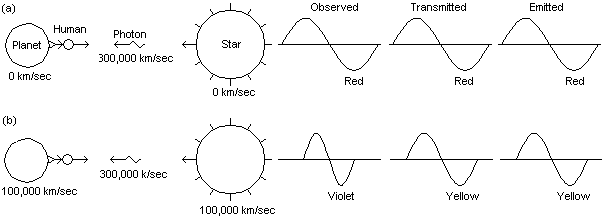Fig 5 The energy in each photon is related to its wavicle-frequency relative to the space continuum     Most energy is composed of vibrating wavicles. When energy wavicles travel at high speed relative to the space continuum there is a Doppler  type effect that occurs. In Fig 5 there are two diagrams, one with the star and planet at rest relative to the fixed space continuum, and a second with the star and planet moving relative to the space continuum. The star, planet, and the human observer are made of matter that are really just a collection of vibrating wavicles. When matter is forced to increase its speed relative to the space continuum, the vibrating matter wavicles are forced to vibrate quicker as well. This is the reason matter gains a large amount of relativistic mass  when it travels near the speed of light. Electrons have been accelerated to near the speed of light in particle accelerators. Some of these electrons have more than 1,000 times as much relativistic mass than their normal rest mass.     In the first diagram there is a single photon wavicle being emitted from the star. Imagine that exited atoms on this star emit photons of red light, depicting a cold dying star. If a single atom emitted a single photon in the direction of the planet, it would leave the star and travel at the speed of light relative to the space continuum. It would travel as a red photon relative to the space continuum. When the photon arrived at the observer on the planet, the observer would see the photon as the same red light relative to the eye of the observer which is at rest relative to the space continuum.     In the second diagram the same dying star of the first diagram has been accelerated up to a high speed so that it is now traveling to the left at 100,000 km/sec relative to the space continuum. The observer on the earth has also been accelerated and is moving right at 100,000 km/sec relative to the space continuum. Now when a single photon is emitted from the star it is not emitted as a photon of red light, rather it will be yellow light. Yellow light is vibrating at a faster rate than red light. Because a photon is really a vibration, as the atom moves at 100,000 km/sec relative to space continuum during an emission, the vibration it is emitting is compressed. The photon that is emitted is vibrating at a higher rate. When the yellow photon arrives at the human observer on the planet, the vibration is once again compressed because the observer is traveling at 100,000 km/sec towards the photon relative to the space continuum. Therefore the observer would see violet light. Photons that vibrate faster contain a larger amount of energy and relativistic mass.     The Doppler effect is much more difficult to utilize when analyzing energy wavicles than when dealing with sound waves. When a train travels along the tracks and sounds its horn, the frequency of horn vibrations do not change significantly in relation to how fast the train is traveling. This is because the horn vibrations are related to the space continuum of space, while the sound it produces is related to the air molecules. When we consider light however, both the light as well as the atom which is producing the emission, are both traveling in relation to the space continuum and change properties the faster they travel. Therefore the above examples are quite simplistic interpretations of the Doppler effect.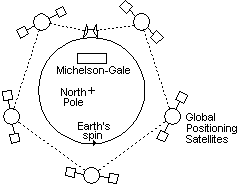Fig 6 The coriolis effect can be explained as the earth spinning in a fixed space continuum     Einstein popularized a certain relative system of measurement which could be called the Observer measurement system. Because of the Doppler effect that occurs when energy wavicles travel in the universe, and because of the speed of light, measuring tools, clocks, and mass all change as an observer moves relative to the space continuum. An observer...is considered to be a human or an instrument that is measuring the event. So it is impossible to get an absolute measurement of distance, time, and mass in relation to the space continuum. So in the Observer system, time is based on clocks the observer has which vary their speed depending how fast they travel relative to the space continuum. Distances in the universe are based on how quickly a ray of light can travel from the distant event. Measurements of mass become only comparisons with other frames of reference.     Another system of measurement that is just as valid as the Observer measurement system is the Absolute measurement system. In this system, all measurements are considered to be relative to absolute geometric coordinates. Einstein refused to think in terms of an Absolute system that I am sure he must have understood existed. He believed that the Observer system was only valid because it is the information that an observer receives . If however we only think using an Observer system, things get mighty confusing. It is like flying in the clouds with no instruments. It is the reason why few people understand what the theory of Relativity is all about. Even today however, this relative Observer measurement system is the only way we have of measuring events in the universe. But does this mean that there is no absolute fixed space continuum of space? It turns out that there have been tests done which can determine that the earth is most likely traveling in an absolute space continuum. Michelson and Gale first showed this in 1925 when they sent beams of light in opposite directions in a circular path of evacuated pipes. A more modern test has been done with Global Positioning Satellites. Two short bursts of light signals are sent around the earth in opposite directions. A difference of time is recorded. This is called the coriolis effect. Such a test clears up some misconceptions about the speed of light. It is often thought that the speed of light will always be measured as being constant relative to an observer. This is not true, a fact that Einstein even pointed out. Some measurements of the speed of light will show that its speed is always constant relative to the observer and other types of tests will show it is not at all. In reality, tests and careful analysis will show that the speed of light must be constant relative to an absolute space continuum only. If we are traveling relative to this space continuum however, our measuring instruments will be skewed in different ways depending on how the tests are done.     We have a dilemma. It seems there is a fixed space continuum but there is no way of measuring to it. To properly calculate events in the universe we must relate these initially to an absolute system because that is what light speed is constant relative to. The best solution to this dilemma is to start by understanding the theoretical model of an Absolute measurement system, then relate this to an Observer system. It is the way that most scientific measurements are understood and the method of measurement that is used when calculating distance and time with maps. It is known that a map is a small scale of what the real event will be. It is possible to plan the trip theoretically based on calculations or the scale of the map. The Absolute measurement system is based on an outside theoretical observer in space measuring at what speed energy travels relative to the space continuum without taking into account the speed of light. Though it is not known exactly where the space continuum is fixed at, it is possible to estimate it from celestial movements. Time instead of being fixed to the changing relativistic mass of clocks is based on movements of certain celestial objects in space just as our present time system is. Distances are theoretically calculated to absolute points in space. Mass does increase as energy travels faster but this is calculated relative to the space continuum. The maximum speed of light in a single direction is always the speed of light relative to the space continuum. The maximum separation speed of two energy wavicles is however twice the speed of light.     Einstein also loved to think in terms of a 4 dimensional universe  with the length of time it took for light to travel between events as being a 4th dimension. Present string theories use from 9 to 26 dimensions . These extra "dimensions" are not spatial dimensions of volume however. They are other attributes of what occurs in that space. Most people identify the concept of dimension with the spatial ones and using the word dimensions for these just leads to confusion. It would be better in physics to define dimensions...as a measure of spatial extent, width, height and length in the absolute sense. Other "dimensions" should be called attributes. Of course it is even possible to create make belief geometric models with many dimensions mathematically, but why? It is even hard for most people to visualize models in 3 dimensions. For example in physics and engineering 3-dimensional volume is often separated into three spatial directions of x, y and z for analysis.     Einstein also suggested that space is curved due to gravity force fields that alter the movement of energy traveling through it. This may have been a fine analogy at the time however we presently have identified at least four different force fields that affect energy. Our present day model would need to have four different overlapping warps because each force affects energy in a different way.     The Observer measurement system, 4-dimensional space and warped space are not in fact an integral part of the universe. They are just modeling techniques that some scientists like to use. We can in fact use any model we like. I suggest that an Absolute measurement system using 3-dimensional space is the easiest for most people to comprehend as the "ground floor" for doing calculations.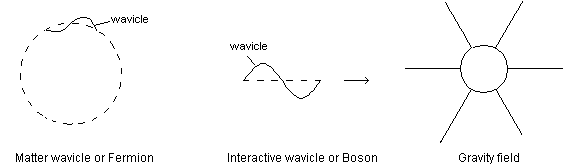Fig 7 Matter wavicles are really just collections of interaction wavicles     Presently force fields and energy wavicles are not very well understood in science . The biggest dilemma is that currently most scientists think that forces are produced by the exchange of interactive wavicles such as photons. In this view it is imagined that the magnetic field is actually a flow of virtual photons emanating from one end of the magnet and being returned in the other. However it is also a well known fact that different types of blackbodies absorb every type of real photon that is emitted. Surely then a blackbody placed in the path of a stationary magnetic field would absorb the virtual photons that were emitted and quickly heat up. At least the magnet would absorb the photons if the virtual photons were anything like a real photon. If virtual photons aren't at all like real photons then they are a completely different kind of entity than real photons with completely different properties. In actual fact force fields do not behave in any kind of way that would suggest that they are made of particles at all.     As was already mentioned, it is possible that the entire universe is composed of at least three layers of entities that can be identified. It appears that there a space continuum which forms a fixed absolute 3-dimensional structure that entirely fills the universe. At present we have no words in our language to describe such an entity that is not made up of any form of matter. It is not solid, liquid or gas, because these are words to describe different formations of atomic particles. We can only use common words such as granularity, cellularity, or metrical to describe the fact that the space continuum must be divided off into very small sub entities. It must have sub entities because the speed of energy as well as force fields that travel through space devoid of matter is very uniform. How would energy wavicles and force fields know how to travel at a certain rate if the space continuum or space were one large entity? Surely photons do not have a sense of detecting their absolute velocity, a computer, and a throttle to control their speed through empty space to such an exact standard as the speed of light. If the space continuum has very small entities, then energy wavicles and force fields could have a uniform speed by jumping from one entity to another or by traveling in relation to these entities. This is somewhat similar to how the speed of sound can be constant by traveling in a medium.     Traveling through the space continuum of space are the force fields. They are called fields because they are spread out into large areas of space. Presently force fields are not very well understood in science . There are four force fields that are thought to exist. The Strong field holds quarks together. The Weak field is responsible for holding together leptons such as the electron. The Electromagnetic field holds together atoms and molecules, is responsible for magnetism and electric fields. The Gravitational field pulls all energy wavicles together. Electromagnetic force fields travel through the space continuum at the speed of light. It is speculated that other force fields may as well. While energy wavicles are vibrating, it appears that force fields are not the result of any sort of vibration. They appear to transfer forces by some presently unknown mechanism. It is not known whether all force fields are the result of one basic type of interaction or if there are four or even more individual interactions that exist. Even thought force fields do not contain energy wavicles, they do contain a certain amount of energy. They can be thought of as extensions of the energy wavicles that drive them. For example a magnetic field can be produced by a flow of electrons in a wire. This magnetic field can in turn produce a flow of electrons in another wire some distance away.     Not all energy is transferred directly through the force fields. Commonly there is an indirect transfer of energy through the creation of energy wavicles such as photons. When the lines of force in a field system move there are often wavicles such as photons that are formed. For example when the electromagnetic field around a radio transmission aerial expands and contracts, real wavicles of low energy called radio wavicles are formed. The moving fields in effect scoop out small wavicles of energy as if out of nothing. In reality though the new wavicle of energy was created by direct transfer of the energy in the transmission aerial through the fields and to the new energy wavicles. If the forces in the field collapse slowly, wavicles with slow vibration equal to the vibrations of the magnetic field will be created. If the magnetic force field collapse quickly, wavicles with a fast vibration will be created.     While field vibrations are large 3-dimensional areas of force, energy wavicles are considered to be individual vibrations of a single separate energy wavicle. Wavicles are often thought of as being very small, in reality they can cover a huge volume of space otherwise our eyes or the tiny aerials of cell phones would not be able to collide with enough of them. Some low frequency photons can be considered to be many kilometers in height or width. For example a curved satellite dish is only composed of wires. Wouldn't photons just travel through the mesh if they were tiny particles? In fact the photons are too large for them to go through the mesh.     Energy wavicles likely travel forward at a maximum speed of 300,000 km/sec relative to the space continuum, which is called the speed of light. It does not appear that the vibrations of the wavicles are vibrations of the space continuum entities, rather the energy wavicles seem to be vibrating in their own cell like structures, but in relation to the metrical aspect of the space continuum. Wavicles at times have been described as vibrating strings or membranes. I like to think of them as simply wavicles, which can include any kind of vibrating string, membrane, ball, blob or bubble.     Groups of wavicles can form wavicle chains and wavicle webs. These various collections result in the different properties that atoms and molecules possess. In the present string theory, all the different types of energy particles are described as different frequencies of the vibrating strings. Such a system does not seem possible because the energy of each individual wavicle is already based on the speed of the vibrations of the wavicles. Photons themselves have vibrations ranging from near zero to extremely fast. It is more likely that all the different atoms are rather created by the different combinations and groupings of wavicle chains and webs. There may also be other possible variations within energy wavicles which at present we cannot detect. For example it appears that energy wavicles have different characteristics such as being open ended, closed loops, and twisting vibrations.     The energy wavicles in the universe can be divided into different groups which have different properties. All energy wavicles can be divided into either bosons or fermions . A boson...is a wavicle which can exist in the same spot as another wavicle. A photon is a boson. A fermion...is a wavicle that cannot exist in the same spot as another wavicle. The basic components of matter such as the proton, neutron, electron, and neutrinos are all fermions. Another way of dividing all wavicles is into those that contain entirely matter and ones that don't. It appears that some bosons can contain small amounts of matter. Matter...is energy that can exist even when it is stationary relative to the space continuum. The term stationary refers to the whole of the matter wavicle not moving. The energy is still moving but rather than traveling in a straight line the energy is circulating within the confines of the matter wavicle similar to the illustration in Fig 7.     There have been many movies made and books written in the past about people traveling near the speed of light. The molecules in a person are made from matter and these would contain much more relativistic mass and energy if they traveled at these speeds. When matter travels very fast relative to the space continuum, the wavicles are not traveling at greater than the speed of light. Rather, the vibrations of the wavicles are faster. It is not likely that atoms with wavicles vibrating so quickly and containing so much energy would be stable anymore. Atoms might start decomposing, forming other types of atoms as well as emitting radiation. This is a factor that Einstein did not take into account in his totally relative Observer system. His system was based on relative effects that took place in experiments with light at very low speeds such as in the Michelson-Morley test. Such effects cannot necessarily be extrapolated to be uniform for all types of energy wavicles all the way to the speed of light.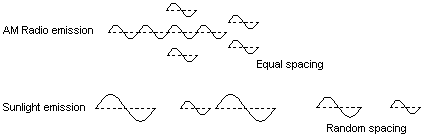Fig 8 We see color based on the frequency of individual wavicles and not on spacing between wavicles     It is understood by most modern scientists that the traveling vibration of energy wavicles is not like ordinary water waves or sound waves. Still textbooks continue to use the standard water wave model to represent energy wavicles. Energy wavicles in fact travel in a completely different way. If a single water wave is generated in the center of a calm body of water, it travels in a spherical pattern from the source. The further away from the source, the weaker the wave becomes. A boat lying far from the source of the wave would experience only part of the energy of the wave as it passes by the boat. This does not happen with the energy wavicle. A single energy pulse can travel for years in space without spreading out if it does not come in contact with matter wavicles. When it is finally absorbed, the entire wavicle will be absorbed into one atom. Not only does the pulse not spread out sideways, the vibration is confined into a small zone as it moves forward.     In Quantum Mechanics, a slightly different model is taught called the wave-particle duality theory. In this theory it is said that an energy particle can behave as either a particle or as a wave, but not both. This is not really an accurate portrayal. In fact an energy particle has particle-like and wave-like properties, but it is neither of the two. Again we do not presently have a common word in our language that describes such an entity. B. Hoffman in fact did coin a new name wavicle for this new kind of object but you will not find it listed in most common dictionaries. Such a new word can describe the real properties of a new entity. It is difficult for us to achieve a clear understanding of the nature of a wavicle because this object is very remote from our present experience that relates to material objects.     It is also a dilemma as to what related words should be used to describe such a vibrating particle, because any terms such as waves, frequency or wavelength can be confusing because they are related to material things. For example the word frequency in the physical wave model usually means the frequency or number of wave crests traveling past a reference point per unit of time. With energy wavicles, frequency does not refer to the number of wavicles traveling by per second but rather the frequency of vibrations of the single energy wavicle. As well, a certain wavelength does not relate to the spacing between different energy wavicles but rather the distance the wavicle travels in relation to the space continuum during one vibration.     Because of this confusion a wave should be defined...as the transfer of energy by some form of regular vibration, or oscillatory motion, in a material medium. Wavicle-frequency is a new word that can be used to describe...the number of vibrations of the energy wavicle per unit of time. Wavicle-length is a new word that can be used to describe...the distance an energy vibration travels forward through the space continuum during one vibration.     Sodium lights produce photons of the same wavicle-frequency and so their light is monochromatic. This does not mean however that the wavicles will be synchronized with each other. Such light will be incoherent because it does not have a regular spacing between photons as shown in Fig 8. Radio and especially laser wavicles on the other hand can be coherent as well as monochromatic. This is why laser light is so powerful. The vibrations of the photon wavicles are completely in step. This is why radio wavicles can induce a current in an electric wire, whereas infrared light wavicles cannot.     When the eye sees a yellow light it is not interpreting the color yellow based on the spacing between photons but rather the wavicle-frequency of the photons. The amount of energy in each photon is called the boson energy expressed in joules/wavicle. The amount of energy contained in each photon wavicle is exactly proportional to the wavicle-frequency. The hotter an object is the larger will be the boson energy of the photon emitted and the higher will be its wavicle-frequency. The boson energy of red light is about half as much as a violet light. The boson energy of a radio photon is very much less than an X-ray photon.     If two separate waves in the ocean are produced in such a way that they are traveling in the same direction and phase, they would reinforce each other and form a new single larger wave. This would be equivalent to forming a new photon of larger boson energy which would represent a different color. While this can occur with water waves, light wavicles cannot be joined together to form one larger one or split apart unless they are absorbed by matter and re-emitted. When more light wavicles are concentrated on a surface it is only the brightness that has been increased. For example it is possible to use a magnifying lens and concentrate light from a large area into a point. The brightness of the light increases, but not the color or boson energy of the wavicles.     Analyzing how wavicles travel through prisms, bend at the edges of a diffraction grating or are polarized can be complicated. Because the energy wavicle is vibrating, many phenomena such as interference and diffraction can occur just as they do with water and sound waves. There are many similarities, but there are also many differences as well. One of the largest differences is that the wavicles are so incredibly small. There are so many events happening per second, it is hard to imagine. When a wavicle comes close to an atom, how will it be deflected? The wavicle is not hitting a flat surface, but an irregular surface composed of the bumpy parts of the atom and is being deflected by the varying force fields that surround the atom. It is impossible to tell which direction the deflection will be because of the many unknown and changing events within the wavicle that cannot be measured during an experiment. Quantum Mechanics therefore suggests that microscopic events are based on chance and probabilities. Einstein didn't think this could be right, and he was correct. The wavicles are obeying the law of physics for microscopic wavicles, it is just that scientists do not have equipment that can measure such a tiny individual event.

 10. EXTERNAL ENERGY vs INTERNAL ENERGY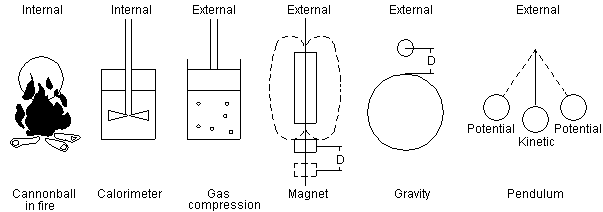Fig 9 Internal energy and external energy forms     If a cannonball, as shown in Fig 9, is heated in a fire it will become hotter but it will not take off out of the fire and leap into space. Why not? The energy that was added was thermal energy. The energy added increases the velocity of the molecules, however the molecules are traveling in all different directions and this does not result in any forward movement of the collection of molecules. This type of microscopic energy is called multidirectional energy. If energy was added to the cannonball by a charge of gunpowder in a closed cannon, the expanding hot gases would be made more directional and so the cannonball would come flying out of the bore of the cannon. The cannonball flying out of a cannon would contain both multidirectional and directional forms of energy. The cannonball however does not contain some molecules that are only traveling forward and some that are multidirectional. The two types of energy are superimposed on one another. In a cannonball flying through the air, though the molecules may be traveling in all directions, the net direction of all of them is forward. It is difficult to understand how directional energy could be stored in the same wavicles as multidirectional energy. Why would the two forms not get mixed up? Why would the directional part not slowly become multidirectional? This does not occur because the energy wavicles do not change direction until acted on by some outside force. There would need to be a directional force canceling out the directional part. Even though the energy wavicles are moving in all directions, the wavicles energy and direction is still perfectly accounted for in the process. If there is a mean direction in the sum of all the multidirectional energy, this will be conserved.     In science it is beneficial to split up energy in the universe into three types, external energy, internal energy, and nucleus energy. External energy  in a very generalized way is directional energy and internal energy is multidirectional energy. There are exceptions though. Because external energy is always stored in the same system as internal energy, it is sometimes hard to identify what is the part that is external energy and so some rules need to be made. Two common forms of external energy are used as the reference, the directionally oriented motion energy such as is contained in the cannonball's forward movement known as kinetic energy and the directionally oriented energy such as is contained in the hoisted block of granite which is a form of potential energy. If other forms of energy can be entirely converted into these two types in a simple one-step mechanism, they are also included as forms of external energy. For example if a gas is compressed in a cylinder the molecules get hotter and contain more motion energy of the molecules, but if the gas is allowed to expand again quickly, the piston will return back to the starting point. Theoretically the compression and expansion would not result in any loss of external energy. The external energy that was stored was in a system of multidirectional energy but it is called external energy. External energy...is the directionally oriented motion energy or multidirectional energy that can theoretically be entirely converted to directional energy in simple one-step mechanisms. Internal energy...is the multidirectional energy contained in the molecular and smaller motions that cannot entirely be converted into external energy or is more difficult to convert into external energy. The nucleus energy is the energy contained in energy wavicles that cannot typically be converted into either internal or external energy. For example it does not seem possible under normal circumstances to convert any of the energy contained in neutrons or protons into thermal energy or electric energy other than a small amount of nuclear energy.     In Fig 9 both the cannonball in the fire and the water in the calorimeter represent forms of internal energy. These are forms of multidirectional energy that cannot be entirely converted into external energy. The energy stored in the iron bar due to the magnet and the energy stored in the cannonball due to the earth's gravitational field are examples of external energy because the energy was added and stored in a directional way. The pendulum alternates between two forms of external energy, potential energy and kinetic energy, both of which are directional forms of energy.     External energy is very valuable because all forms of external energy can be entirely converted into other forms of external energy theoretically. Unless perpetual motion is possible it is impossible to convert most forms of internal energy entirely into external energy. It is also impossible to convert many forms of internal energy entirely into other forms. 11. FIRST AND SECOND LAWS OF ENERGY     Scientists are quite convinced that the property defined as energy cannot be destroyed in the universe. Energy can be thought of like dollar bills. If a country only prints X amount of dollars, then different people and companies will own and trade these dollars, but the total amount will never change. Presently this is called the Law of Conservation of Energy in physics or in engineering is called the First Law of Thermodynamics. It is more appropriate to call this the First Law of Energy...einstein energy can neither be created nor destroyed. The sum of all forms of energy remains constant in the universe. Einstein energy...is the total of all forms of energy in the universe or in a specific system  which will be described in more detail in Chapter 13.     If any one type of energy could be converted entirely into any other specific form of energy, then perpetual motion would likely be possible. Though there is no basic scientific principle that does not allow perpetual motion, scientists feel that it is not possible. In engineering, the Second Law of Thermodynamics states that...thermal energy can never be entirely converted into external energy. Thermal energy is a form of internal energy, which is the motion energy of the molecules. There are however other forms of internal energy such as chemical energy that also cannot be completely converted into external energy. Even a fuel cell theoretically cannot entirely convert chemical energy into electricity, which is a form of external energy. Therefore all types of conversion of internal energy into external energy are limited by the Second Law of Energy...different forms of energy cannot necessarily be entirely converted into other forms of energy . Obviously this law is not very explicit because it will take several pages of words to describe what the limitations on energy conversion are. It is simply a warning of what to expect. 12. SECOND LAW EVALUATIONS     A Second law evaluation...is a calculation of the maximum amount of external energy that could be extracted from a system based on a certain reference atmosphere. A reference atmosphere...is a body of matter such as the earth that energy can flow into during a conversion of internal energy to external energy. In engineering the second law evaluation is also called an exergy evaluation or availability evaluation, but this is generally a more specific type of evaluation involving only clausius energy, which will be introduced in Chapter 13. If a second law analysis were done in biology, this would for example entail a calculation of the maximum amount of muscle energy that could be obtained from a certain amount of food energy. Not all the food can even be theoretically converted into muscle work. Food is a form of internal energy and cannot be entirely converted into muscle energy, which is a form of external energy. Then there are other irreversible losses in the muscle itself that result in even less muscle energy being produced than the theoretical amount calculated. In Chemistry it is the internal energy liberated in a reaction that is of primary importance rather than how much external energy could be extracted. Second law concepts however are the basis of why chemical reactions will occur. Even so second law analysis is not always part of a standard biology or chemistry course even in university. It is only first law evaluations that are generally done.     Physics and Mechanical engineering are typically the disciples where second law evaluations are generally taught. But while chemistry uses second law evaluation terms like helmholtz energy and gibbs energy, mechanical engineering uses totally different terms like availability and exergy. The chemistry terms such as chemical energy or helmholtz energy are referred to here as the plural energy system. The mechanical engineering system which uses the terms energy and exergy on the other hand is referred to as the singular energy system. Exergy...is considered to be the maximum amount of external energy that could be drawn from energy based on a certain reference atmosphere. Exergy is not considered to be a form of energy but a designation of the quality of energy. Availability is a word that means the same as exergy.     Both the plural energy system as well as the singular energy system were started in the mid 1800s. Scientists like Gibbs and Helmholtz started the basis of the plural energy system while others like Clausius developed the singular energy system. The plural energy system as described in Chapter 13 has several inherent advantages over the singular energy system and is what is used in this booklet.     The first biggest advantage of the plural energy system is that everything is a form of energy. This is a word that is already well recognized. Hardly anyone in the general population has ever heard of exergy. They don't know to even associate this word with energy.     A second key advantage of the plural energy system is that it allows the present exergy evaluation to be broken into at least eleven divisions; external, kinetic, potential, electrical, mechanical, expansion, helmholtz, gibbs, free, clausius and bound energy. This makes a second law evaluation more precise as each of these are not really equal in worth when comparing realistic processes. Many of these subforms of energy listed in Chapter 13 are already used in chemistry. The singular energy system sometimes uses similar subforms of exergy, but words for these subforms are even less recognized than the word exergy.     The third advantage of the plural energy system is that all subforms of energy can be added up in the same column, equation etc. They can also be identified as units of energy. In the singular energy system the two entities must be kept separate because energy and exergy are not considered to be the same. This is because energy and exergy always overlap each other and do not add up to the total einstein energy.     Proponents of the singular energy system maintain that forms of clausius energy in the plural energy system are not really energy because they are not conserved in the universe whereas energy is. In reality though, most engineers that use the singular energy system also use terms such as chemical energy, nuclear energy, kinetic energy, and potential energy. So it can be seen that the singular energy system uses names from both systems, which are not really compatible. In reality there is really only one form of energy that is conserved in the universe and this is named einstein energy in the plural energy system.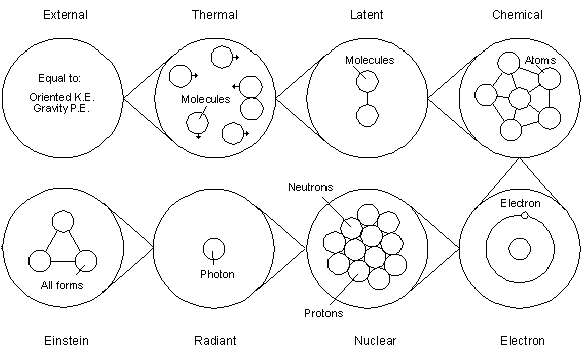Fig 10 Einstein energy can logically be divided into different forms     It is beneficial to have a proper naming system that covers all the basic types of different energy in the universe. This is because it is often difficult or impossible to convert certain types of energy into other different forms. The system of energy proposed in this booklet is based on the plural energy naming system where all the different types of energy are two word forms such as nuclear energy. The basis of this two word naming system is borrowed from chemistry. Many scientists over time have tried to establish single word names like enthalpy, essergy and anergy for the different forms of energy but this is not necessarily desirable as few people would recognize them as forms of energy. It is also confusing for some forms of energy to be two words such as internal energy while others are not such as enthalpy. As well there are not enough different names that sound similar to energy that can be used when many forms are used. To be consistent, these single word energy names were converted into two word names in the plural energy system.     The plural energy system is shown as a series of bar charts in Fig 11. Most of the names are presently used in some discipline of science however some are new. The 28 most popular forms of energy are shown in three separate charts. The first chart shows relativistic forms of energy, which will be discussed in Chapter 16. The second chart shows the 17 simple forms of energy that are the most widely used. The last chart shows the complex forms of energy which are used in more complex energy calculations.     At the head of the simple forms of energy chart is einstein energy which is...a new term for the concept of the total energy in the universe or a particular system. When referring to the fact that energy is conserved in the universe it should be mentioned that it is einstein energy that is conserved, because other forms may not be. All einstein energy can be logically divided into either external energy, internal energy, or nucleus energy, which was defined in Chapter 10. External energy is logically divided up between the major forms of kinetic energy and potential energy. Kinetic energy...is the external energy possessed by a body because of its motion. Potential energy is the external energy possessed by a body because of its position. There are many subforms of external energy like gravitational energy and magnetic energy, which are not listed in the chart because these are commonly just identified with potential energy. Electrical energy and mechanical energy are listed however because it is often desirable to keep these forms of energy separate. Expansion energy such as that contained in high pressure air in an automobile tire is also listed because it is an important hybrid form of external energy. It has many of the characteristics of internal energy but is classed as external energy. Often all forms of external energy are grouped together in one category. This is because all forms of external energy can theoretically be entirely converted into each other so differentiating is not so important.     Internal energy is logically divided up between eight different types that are the most used. Thermal energy...is the motion energy of the molecules. Latent energy...is the energy stored in the atom due to the van der Walls forces between the molecules in a substance which is stored or released when undergoing a change of state, such as ice changing to water or water to steam. Solution energy...is the change in energy of a solution when a certain amount of solute dissolves in a solvent. Chemical energy...is the energy stored due to the bonding of the atoms in the molecules. Electron energy...is the sum of both the motion and position energy of the electron wavicle. This is not the same as electrical energy which is a form of external energy. Radiant energy...is the energy contained in bosons such as the moving photon wavicle. Nuclear energy...is the energy stored due to the bonding of the particles in the atoms nucleus, only the energy of a nuclear reaction is considered. Nucleus energy...is the energy stored in the nucleus of the fermion such as the atom.     In present textbooks, chemical energy is described as the vibrational energy between the atoms or molecules. This makes it sound as if the atoms are vibrating back and forth. This is not really true. If this was the case, the molecules would quickly loose their energy to the thermal energy system around them, and the molecules would soon disintegrate. The vibrational energy that is being referred to is really the wavicle vibrations inside the building blocks of the atom itself. In Chapter 15 this will be discussed in more detail.     Radiant energy is considered another hybrid form of energy because while most radiant energy is unorganized and cannot entirely be converted to external energy, other forms such as radio wavicles are highly organized and theoretically can be entirely converted to external energy.     Caloric energy...is a new term which represents the amount of internal energy that will flow between two reservoirs . Caloric energy generally cannot be completely converted into external energy. It can be split up into two parts for analysis. The helmholtz energy...is the part of caloric energy that could be converted into external energy in a future process. The bound energy...is the part of internal energy or caloric energy that cannot be converted into external energy.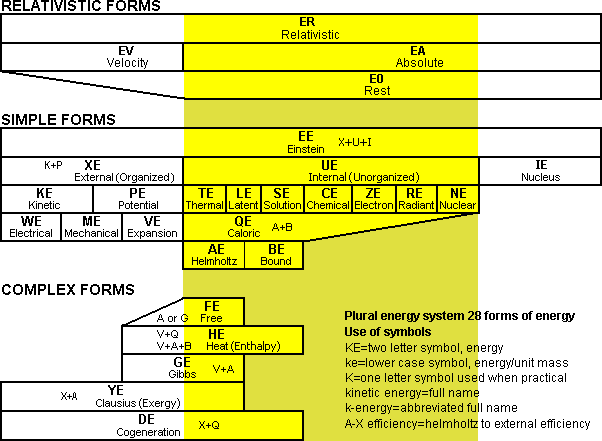Fig 11 Popular 28 forms of energy in the plural energy system shown in a bar chart     There are another seven complex forms of energy shown in the third chart of Fig 11. Substances usually contain mixtures of external energy and internal energy. Scientists have developed many terms for combinations of these two types of energy. The reason these combinations are used is that it is often too much work to separate an internal or external energy addition.     For example when thermal energy is added to air in a room, this thermal energy results in the air expanding. It does work against the atmospheric pressure. This is external energy or more specifically expansion energy that is stored in the expanded air volume. The rest of the thermal energy goes into elevating the temperature of the air, a form of internal energy. Because this thermal energy is not added with the intent of extracting any work, all that is generally desired is to know how much thermal energy needs to be added to raise the temperature of the air in the room. The energy that is stored in the air of the room is called heat energy in this booklet. It is often called enthalpy presently. It contains a mixture of internal and external energies.     Gibbs energy...is composed of helmholtz energy plus expansion energy. Free energy...is a generic term that refers to either helmholtz energy or gibbs energy. Clausius energy...is composed of external energy plus helmholtz energy. Generally in the singular energy system mentioned in Chapter 12, when it is stated that a certain process has a certain exergy, this is equal to an amount of clausius energy. Cogeneration energy...is a new term that is composed of external energy and caloric energy. It most often represents the combination of electricity and thermal energy available in a co-generation power plant.     When dealing with combinations or subclasses of external or internal energy, there is often little concern with whether the energy is chemical or radiant etc. At other times though it is necessary to consider the various forms of energy such as chemical or thermal separately.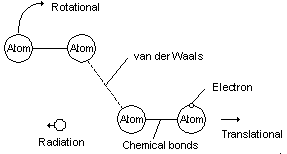Fig 12 Places where energy is stored in internal energy 14. UNITS     Because there is such a large difference between the behavior of external energy and internal energy it would be best to use different units for each. This would make it easy to differentiate between external or internal energy. As was just shown however, calculations very often involve mixtures of the two, which can rightfully be added together to comply with the First Law of Energy. The solution is to use one basic unit of energy but tag the energy type to separate them. This is similar to accounting where the dollar is the common unit of money. In a corporation though some money is in the form of actual currency, other money is in the form of land, buildings, shares and even the value of patents. These cannot all be exchanged easily for each other but in the books they are all valued in dollars. Note that because tags are used extensively in this system, when there is multiplication used in an equation, the multiplication symbol, which is a small centered dot "·" should always be used. The following equation uses tags such as XE, QE, AE and BE after the amounts of energy. The abbreviations are listed in Fig 11 or at the end of the booklet. 100XE=100QE=30AE + 70BE     In science, the metric system is the preferred system of units around the world. In the United States, engineers often use the old English system of units, which is now named the American system. In this system the ft-lb is the unit of external energy and the BTU is the unit of internal energy. The older metric system also had such dual units. The joule or newton-meter was considered the unit of external energy and the calorie was the unit for internal energy. When doing calculations for energy conversion devices such as gas turbines, it is too cumbersome to use dual units for energy. Usually one or the other was picked. This is why presently the more preferred SI metric system uses one unit of energy.     In the SI metric system, the joule or kilo joule is considered the standard unit for energy where 1 joule (J)=1 newton-meter of energy and 1 kilo joule (kJ)=1000 joules. SI...is a French term that stands for International System of units. It is the system that is preferred in engineering and physics and is making quick inroads into chemistry and biology. The joule is the preferred unit of energy in the SI metric system as opposed to the calorie.     However, though the joule is the standard for energy in the SI metric system, the watt-hour or kilowatt-hour is fast becoming the unit of choice when working with energy conversions where electrical or mechanical energy are involved.     In this booklet the proposed units for temperature in the metric system are either degrees Celsius written °C or degrees Kelvin written °K. As of 1967 the presently accepted symbol for Kelvin is K minus the degree symbol. This however is confusing as Celsius is most often expressed in degrees and Kelvin is not. Also K can be mistaken for K, the proposed single character symbol for kinetic energy. Because the English alphabet has a limited amount of letters to be used as symbols, standard character set designations like the degree symbol can be used to further identify a unit. The degree symbol can easily be typed on a PC computer by holding down the Alt key while typing 248.

 15. TEMPERATURE OF INTERNAL ENERGYFig 13 Internal energy has varying amounts of energy per wavicle     Energy is a two dimensional property of force times distance potential. The previously mentioned First Law of Energy says nothing about the ability of different energy wavicles to generate a certain amount of force. As was already mentioned, all forms of external energy can be theoretically converted entirely into other forms of external energy. For example a block and tackle can be used to multiply the force that can be delivered. By this means someone can pull with a 100 kg force and raise a block of 2000 kg. Internal energy cannot be converted in this way. It is like the tow truck shown in Fig 13. Imagine that the tow truck has enough gas in the tank to provide 100 units of energy. Could it be assumed that the tow truck can pull a train up a very steep grade with a total of 10 units of force but only for a very short distance of 10 units? Not really! The tow truck may not have the capacity to provide this amount of force.     Previously a new term "boson energy" was introduced. This is the amount of energy that a matterless boson like a photon has. Temperature is a term  that will be used in this booklet to describe the amount of energy that all types of internal energy has. We could use Joules or BTU as a measure of the amount of energy that each photon contains. This would be a very small fraction of a Joule such as 1.23 x 10-23 joules, which are very small numbers indeed. It is simpler to use a scale that merely represents the amount. We already use the scale called temperature to measure thermal energy. This represents the average collision energy between molecules. Real photons are created during these collisions, which are equal in energy to each collision as shown later in Fig 17. Therefore radiant energy can already be thought of as having a certain temperature. This thinking can be extended to represent the amount of energy in all types of photons and all types of internal energy. Units of temperature such as 1000°K are easier to visualize and compare. Temperature...is a scale that represents the amount of energy per wavicle of different forms of internal energy and is equivalent to the maximum temperature that would result if converted entirely into thermal energy. To avoid confusion we can use terms such as thermal temperature and chemical temperature to avoid confusion.     Types of energy such as chemical energy and nuclear energy represent a certain amount energy that can be liberated per molecule or atom. For example when a hydrogen atom bonds to an oxygen molecule, not as much total energy is required in the newly formed water molecule as in the separate hydrogen and oxygen molecules. A certain amount of energy can be released. When the hydrogen-oxygen bonding occurs, the excess energy under ideal conditions can be released as a single package of energy for each newly created bond. In other words the excess energy is not dribbled out in multiple randomly sized amounts of energy. This single package of energy may not ever form a real photon that leaves the confines of the molecule. It is however an amount of energy which has been released from the atom or molecule. So it is rather referred to as a virtual photon. Such photons have a very limited range in distance that they can cover before they become real photons. In many chemical reactions, virtual photons of 10,000°K or more can be created. In nuclear reactions photons containing even larger amounts of energy can be created of around 2,000,000°K. It is not possible however to take a certain quantity of thermal energy with a temperature of 300°K and convert this totally to chemical energy of 10,000°K or nuclear energy with 2,000,000°K temperature. In fact under normal circumstances none of the thermal energy could be converted into nuclear energy. This is because energy does not flow naturally from a low temperature to a higher temperature. The direction of the microscopic energy flow is caused by the way photons are emitted as will be shown in Chapter 19, 20.     When working with temperature in energy calculations it is best to use degrees Kelvin or °K which is the absolute temperature scale. This absolute scale uses 0°K to represent zero energy of any form of internal energy. The Celsius or °C scale is only used to represent thermal energy. It uses the same energy per wavicle as degrees Kelvin but starts the scale at 0°C which represent the freezing point of water at normal conditions on earth. This 0°C is equal to 273.15°K. It is possible to have a very cold substance that has thermal energy of 0°K but still contains chemical energy of 10,000°K and nuclear energy of 2,000,000°K. 16. TYPES OF MASS AND ENERGY     Presently the word mass in science is usually defined as...the quantity of matter in a body. Photons do not contain matter and so are said to contain no mass and are called massless.     But this has not always been the definition of mass. Writers in the post Einstein period of 1920-1950 such as Eddington almost always considered the term mass to be a shortened form for the relativistic mass that an object possessed.     When energy travels relative to the metrical space continuum, it is the Doppler effect that produces a resistance to change in velocity. If a cannonball is accelerated out of a cannon, the energy required to accelerate it is added to it's total energy content. This total energy content is called the relativistic energy and the mass of this energy is called the relativistic mass.     Many modern writers such as Stephen Hawking also use mass to mean the relativistic mass . When they write about the more specific mass of matter at rest with an observer, they use the words rest mass or invariant mass. Physicists have argued about which is the right method for over 80 years.     To properly come to a conclusion about how mass should be defined it is necessary to understand why we need the word mass to begin with. Presently the concept of mass is usually used to calculate the inertia and gravity effects of bodies of matter. It turns out however that inertia and gravity effects are really related to how much energy a body possesses. So for example gravity acts on beams of light as well as bodies of matter. So it would seem appropriate that all forms of energy should have mass. Looking at the issue even deeper it turns out that we don't really need mass at all. We could simply use the energy content.     It is however usually more convenient to use mass than use the amount of energy for one simple reason. Take again the example of the cannonball that is accelerated out of the cannon. It gains a very small amount of external energy. This gain in external energy usually is very very tiny compared to the total energy locked in the atoms of the cannonball. If we use a mass that is a very small ratio of the energy content, then the gain in external energy compared to the mass is now more similar. Also we don't have to define which type of energy we are talking about in each case. We know mass represents the energy of the matter in the cannonball and the gain in energy due to an increase in speed represents the external energy. It turns out however that in more complex Physics calculations it is very beneficial to use many different forms of both mass and energy.     In the Absolute measurement system mentioned in Chapter 7, it is desirable to subdivide both "mass" and "energy" into four two word subtypes. Absolute mass...is the total mass of matter wavicles that are at rest relative to the space continuum. This is the mass listed in the periodic tables. Rest mass...is the total mass of matter wavicles that are at rest relative to the observer which may be traveling at various speeds relative to the space continuum. This is a specific form of the relativistic mass. Relativistic mass...is the total mass of energy wavicles that are traveling relative to the space continuum. Velocity mass...is the increased mass of all matter wavicles as they travel faster relative to the space continuum, it is the difference between two values of relativistic mass at different speeds. In reality we will never know what the absolute mass of matter is. Because it is likely that the earth is traveling very slowly through the space continuum compared to the speed of light, we can closely approximate the absolute mass by using rest mass on earth. Mass is now defined as...a value that is proportional to the amount of energy in an entity.     From a grammatical viewpoint, it is safer to use the shortened word mass to represent relativistic mass, which covers all forms of mass. In reality it is more precise, especially when relating to the more complex aspects of mass in science, to write or speak using the full two word descriptions at all times. This is because people will never come to an agreement of what the single word mass should mean. The four equivalent forms of energy represent the energy contained in each of the four types of mass. These have been shown previously in the charts of Fig 11. Note that relativistic energy is exactly the same as einstein energy. Why two terms for what is really one form of energy? It is to differentiate between when we are specifically dealing with relativistic effects and when we are not. MA = absolute mass, kg M0 = rest mass MR = relativistic mass MV = velocity mass EA = absolute energy, joules E0 = rest energy ER = relativistic energy EV = velocity energy v = velocity of mass in m/sec relative to space continuum c = velocity of light, roughly 300,000,000 m/sec relative to the space continuum     When a cannonball is stationary relative to the space continuum, it contains an amount of absolute mass. When a cannonball is stationary relative to an observer, it contains an amount of rest mass. When the cannonball is accelerated through the air, it's total relativistic mass increases. In physics it is usual to only calculate the increase in the relativistic energy which is the increase of it's velocity energy.     It is possible to calculate the extra amount of velocity energy that the cannonball contains when it is traveling at low speeds relative to the speed of light by using the well know physics formula Eq (1). Note that velocity energy can relate to both thermal energy and kinetic energy.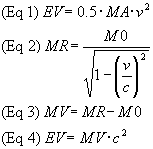Because the cannonball has gained energy, it has also gained mass. It is possible to calculate this mass through a type of relativistic Doppler formulae, which is called the FitzGerald relationship, as shown in Eq (2), which equates the velocity of the rest mass to the new larger relativistic mass of the cannonball. The gain in velocity mass can be calculated with Eq (3) which subtracts out the rest mass. If this mass is plugged into the well know Einstein Eq (4), it will be found that the energy calculated will be identical with what is calculated with Eq (1). Note that Eq (4) does not look like the usual Einstein equation because it uses the more exact symbols for energy and mass.     This can all be confusing at first. Basically all four forms of energy are related to their own specific forms of mass with variations of Einstein Eq (4). In other words, the rest mass of matter is related to an amount of rest energy, or the velocity energy is related to its gain in velocity mass using Eq (4). When comparing how much energy is gained due to other forms of mass there are different ratios that apply. For example when rest mass such as in the cannonball is accelerated, its rest mass is related to its velocity energy gain by Eq (1). The photons entire energy however is velocity energy, and its energy is related to its velocity mass by Eq (4). The relativistic mass of matter compared to its rest mass is calculated by Eq (2). It seems odd that the gain in velocity energy of matter compared to its absolute energy at low speeds is only one-half of what it is at high speeds. This may be due to wavicles having a different Doppler effect when they are traveling in a straight line relative to the space continuum vs traveling in a circular fashion.     Figure 13 shows this difference just mentioned in an object such as a cannonball. Note that at low speeds the typical formulae Eq (1) applies. At a certain higher speed the figure 0.62 would have to be substituted for 0.50 in the formulae.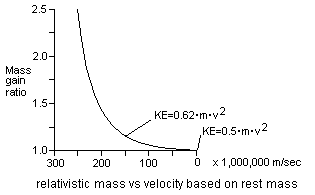Fig 14 The formulae to calculate the velocity energy changes with speed     When a nuclear fission explosion occurs such as in an atomic bomb, the original collection of matter wavicles of the uranium are rearranged into a new collection of matter wavicles. There is a small amount of mass left over that cannot be utilized in the formation of the new collection of wavicles. This small amount of mass results in a great deal of energy being liberated, largely in the form of super high energy photons. The amount of energy liberated can be calculated with a variation of Eq (4) using absolute mass and absolute energy. Because both matter and photons have both relativistic mass and relativistic energy, it is not proper to say that mass is converted into energy in the system suggested here. It is better to say that a certain amount of matter was converted into other forms of matter and released excess energy in the process .     The amount of absolute energy stored in the absolute mass of a 1 gram piece of aluminum is 90,000,000,000,000 joules. This is enough energy to power the average house at a normal average electricity use of 1 kw for 2,854 years. Most of the energy contained in the aluminum however could not ever be converted into electricity. Even if one obtained a 1 gram piece of pure uranium, the maximum amount of energy that could be liberated from it would be about 1/1000 of its absolute mass. This is the nuclear energy of the uranium. As well, not all of the nuclear energy can be converted to external energy.     Just as energy is conserved in the universe, mass is too. Therefore we also can have a First Law of Mass...mass can neither be created nor destroyed. The sum of all forms of mass remains constant in the universe. There is also a Second Law of Mass...different forms of mass cannot necessarily be entirely converted into other forms of mass.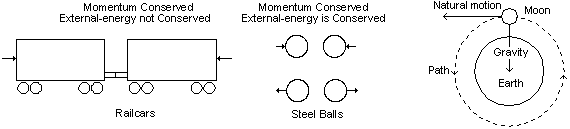Fig 15 Momentum is different than energy     As was mentioned in Chapter 6, there is a system that energy travels in composed of the space continuum. A key characteristic of the space continuum is that energy travels in straight lines if there is no interference from other energy wavicles or force fields. Why it does so is not understood presently. Besides wanting to travel in a straight line, another property of the space continuum is that energy wants to travel in the same direction and at the same speed. Both of these properties can be considered to be the momentum of the energy. Energy wavicles have momentum because if they change in velocity relative to the space continuum, the Doppler effect requires that they change their energy content. When energy is not available or cannot be disposed of, they cannot change in speed.     When objects such as the moon or spacecraft travel around the earth, they want to go in a straight line. Gravity force fields are traveling towards the center of the earth however and so the moon travels in a circle around the earth. If gravity is pulling downwards, then how do astronauts feel weightless? It is because the acceleration due to moving away from the natural motion of space continuum and the acceleration due to gravity are completely balanced off. Not only are they balanced but both of these forces are balanced throughout the entire body of the astronaut. Both inertia and gravity act on every molecule in the body.     In the General Relativity theory proposed by Einstein, acceleration is considered to be the result of the force fields in the universe. Einstein proposed that the reason why gravity and inertia appear to feel the same is because they are equivalent . It appears he believed that they were the same thing. Based on the model presented in this booklet, it appears that both inertia and gravity are the result of the space continuum's effect on energy. It appears that gravity is caused as a result of a force produced by its field and inertia is caused by a movement relative to the space continuum. This view is necessary to account for the way different forces act on energy. Because both the gravity field and travel in the universe are relative to the same space continuum, the effect appears to be identical. This does not make them equivalent in my view, however both effects could be considered to be very closely related.     When force fields are pulling or pushing on energy wavicles, they create a type of artificial space continuum. Einstein referred to this as warped space. For example imagine a cannonball is placed on top of a table. The moving gravity force field acting on the cannonball is like a flowing river. If one put a raft in a flowing river, it would naturally flow at the river's speed. In a dense fog it would be hard for boys on the raft to tell that they were moving relative to anything else. They might imagine that the river was not flowing and they were not moving anywhere. Force fields are slightly different than the river because force fields result in an acceleration while the river has a steady velocity. The cannonball would not travel at a steady speed on its way down to the floor, rather it would be accelerating.     Mass and energy are conserved in the universe. Does this mean that when the cannonball is lifted from the floor to the table, the relativistic energy and relativistic mass increase in the cannonball? It appears they do. Compared to the gravity force field, the cannonball is accelerating away from the earth while it sits on the table. The Doppler effect results in a faster wavicle-frequency and more relativistic mass. To get the cannonball onto the table, there were energy wavicles such as photons transferred to the cannonball during lifting the cannonball to the table. We can consider the cannonball to have additional rest mass on the table vs on the floor. The proper definition of absolute mass then relates to its mass without considering any outside forces acting on it. As the cannonball falls to the floor, some of the rest energy is converted into velocity energy. On hitting the floor, the velocity energy is converted into thermal energy. This is a form of internal energy. Some of this is radiated out as radiant energy. And so the transactions go on and on. Now if we considered all the interactions between external energy to internal energy, the Doppler effects of all four forces, and the possible changes between the 23 forms of energy, it would be enough to make one's head swim. Even so, we haven't even considered energy wavicles changing from one form to another, black holes, and antimatter. The universe is a dizzying place to be living in.     It may seem confusing at first how an objects momentum could always be conserved. For example Figure 14 contains diagrams of two types of collisions. In the first there are two rail cars traveling in opposite directions toward each other. They collide and lock together. It appears that the momentum and energy of the objects is destroyed. Should they not bounce off each other? In fact the momentum and energy are conserved in the collision. All of the inertia and kinetic energy of the collision is turned to the motion of thermal energy in the process. In the second diagram there are two steel balls colliding. They bounce off each other in the collision and both momentum and external energy are not converted into internal energy in this process. All the inertia and momentum are retained by the external energy. (Eq 5) Momentum=MR·v MR = relativistic mass, kg v=velocity, m/sec 18. ENERGY VS WORK AND HEAT     In currency and accounting systems there is only one form of money stated. Money is either in an account or money is being transferred. In energy systems it is also proper to say there is so much energy at a certain point of a process or that energy is transferred during a process. Presently in engineering and sometimes in physics the term work...is used to represent all forms of external energy transfer and heat...is used to represent the transfer of thermal energy . This system was satisfactory 150 years ago when it was developed. At that time it was mostly the conversion of thermal energy to external energy that was being studied. There are many other types of internal energy transfer that are not covered in this system. Today we are interested in the conversion of many other forms of energy. Presently it is better to use the 28 names of the standard energy naming system to represent the transfer of many types of energy. For example it is more precise to say that helmholtz energy was transferred rather than talking about a heat transfer.     In the present system, work is defined two ways, which can be confusing. In the true physics definition work represents all forms of external energy and internal energy transfer, however in the engineering definition it represents only the external energy transfer. It is better to have a single definition for work.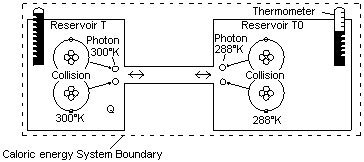Fig 16 Caloric energy is the amount of internal energy that would flow from one system to another     In the gas expansion examined in Chapter 10 there is a type of flow of the multidirectional energy of the molecules from a small space into a larger space. Conversions from one form of external energy to another form of external energy such as this can occur in a single reservoir of energy. Converting internal energy such as thermal energy to external energy is much more difficult and a flow between two reservoirs is required. A reservoir...is considered to be a source of internal energy in equilibrium or at the same uniform temperature. In this booklet the flow between two reservoirs is called caloric energy. This term is not used in present textbooks. Presently it is said that the difference in total internal energy of two systems results in a flow, but it is easier to deal with a single energy rather than a difference of two energies. Carnot described this flow of internal energy to be similar to a waterfall. This waterfall though is not external energy, it is rather a waterfall of internal energy.     If Reservoir T has a temperature that is higher than Reservoir T0, then caloric energy could flow between the two reservoirs as shown in Fig 16. Why does this occur? The flow of internal energy is based on the fact that matter wavicles like atoms and molecules with higher temperature emit more photons per second and larger photons of greater photon energy than matter wavicles with lower temperature. This means that matter wavicles of higher temperature lose energy more quickly than ones of lower temperature. All forms of internal energy can be caloric energy.     The amount of internal energy that can flow is not based on the temperature difference at the start of the flow only, because as the hot reservoir is becoming colder as the heat flows out, the colder reservoir heats up. This is why the ambient Reservoir T0 is always considered to be infinitely larger than the Reservoir T unless stated otherwise. Reservoir T...is considered to be a localized source of internal energy. Reservoir T0...is the ambient reservoir which is always considered to be infinitely larger than Reservoir T. This is the case when the earth is used as the second reservoir. When the Reservoir T0 is much larger than the Reservoir T, its temperature does no change appreciably as energy flows into it. When the word or symbol ambient temperature or ambient reservoir is used, it is implied that the ambient reservoir is infinitely large compared to the other reservoir. A dotted line is drawn around both reservoirs depicting the caloric energy system boundary. The system boundary includes a source of internal energy and the local ambient environment in which the internal energy will flow into. Present textbooks show the system boundary drawn around only Reservoir T because the concept of caloric energy is not used.

 20. HELMHOLTZ ENERGY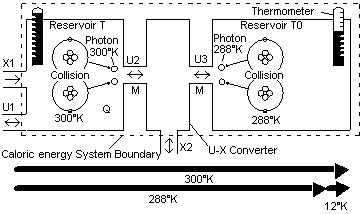Fig 17 The carnot ratio is the amount of helmholtz energy in an amount of caloric energy     The maximum amount of external energy that can be extracted from two reservoirs of truly unorganized internal energy is predictable. The model shown in Fig 17 can be used to analyze such interactions. In classical thermodynamics such a model for thermal energy was based on the molecular kinetic theory, which depicts molecules colliding against each other and gases expanding. The model in Fig 17 is based rather on the quantum model where all forces between matter wavicles are the result of electromagnetic force fields. When two atoms collide they do not merely bounce off each other, the impact of the collision is absorbed temporarily by the atom's electron, which gains energy. This increased energy does not result in a stable orbit for the electron, it wants to shed this energy as quickly as possible. It appears that this is because the electron wavicle has shifted into an orbit where its vibrations are no longer even divisions of the orbital path. Sometimes the atom immediately reabsorbs the energy from the electron into the translational field, which propels the wavicle in the opposite direction. At other times a real photon is emitted, which is radiated away from the atom in the form of radiant energy. Such photons are often quickly absorbed in neighboring atoms, but if the atoms are next to a vacuum such as for example outer space, they could travel for thousands of years with little change.     In collisions, it appears that the atoms come to a complete stop relative to each other and become at least temporarily glued together by the van der Waal forces . Because the atom collisions results in all the atoms' motion being removed, each photon produced represents an energy amount that corresponds to the absolute temperature, not just the difference in temperature between the two reservoirs . It is likely that two identical photons are produced in each collision.     The photon absorption is however different than the photon emission. The photon is not absorbed into only atoms with zero thermal energy, it merely finds any atom to be absorbed into. It would seem that if a 300°K atom could just absorb a few more photons of say 300°K, it could reach 1000s of °K. Alas, soon after each photon absorption, the atom is generally involved in another collision and two photons of 300°K are produced and so it can be seen that the laws of averages or equilibrium apply and atoms with higher than average temperament will be quickly reduced to the mean temperament of surrounding atoms.     It is these differences in emission vs absorption of the system that result in the limited amount of external energy that can be taken from internal energy. In Reservoir T as shown in Fig 17, there are two atoms of 300°K colliding, depicting the average wavicle energy in the reservoir. The photon of 300°K could travel over into Reservoir T0 where atoms are emitting photons of 288°K. In between the two reservoirs there is a 100% efficient solid state material called the U-X converter that absorbs the photon, extracts some external energy and re-emits a smaller photon into the T0 reservoir. It would be possible to only extract the difference in photon energy or 12°K of external energy because of the laws of equilibrium. If more external energy than this is extracted, the photons from the T0 reservoir would be hotter and the net photon flow would be in the reverse direction. The amount of external energy that was extracted was 12 units, the amount of internal energy U2 removed from T was 300 units and the amount of internal energy transferred to Reservoir T0 was 288 units. The 300 units of energy in Reservoir T that would flow to Reservoir T0 represents the caloric energy, the 12 units in the caloric energy that could be potentially converted to external energy represents the helmholtz energy and the 288 units that will flow to Reservoir T0 to maintain equilibrium represents the bound energy.     The above scenario is a description of what occurs with reservoirs of thermal energy. As pointed out in Chapter 15, other forms of internal energy are not the result of molecules bumping into each other. They are due to the bond energy between the different atoms and molecules. So in the case of these other forms of internal energy, there are virtual photons produced in Reservoir T. Sometimes these virtual photons would flow into a Reservoir T0 also made of virtual photons. In other cased the virtual photons would flow into a system of real photons. In many cases such as fuel cells, the virtual photons of the chemical energy flow into the real photon thermal energy system of the ambient surroundings. Obviously this is a very simplistic picture of what is occurring. In all cases however whether the photons are virtual or real, the same ratios of helmholtz energy and bound energy apply.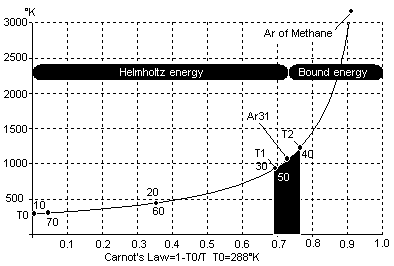Fig 18 The carnot ratio plotted on a graph shows the changing conversion efficiency during a process     The example just mentioned in Chapter 20 however only involves a single photon being converted. Real life energy conversion processes involve millions of photons. Also the temperament of Reservoir T may vary during the process. The carnot ratio Cr...can be used to represent the external energy that could be extracted from a flow of caloric energy at an instantaneous moment such as when a single photon is converted . It can be calculated with Eq (6). The helmholtz ratio Ar on the other hand...represents the external energy that could be extracted during the entire flow of caloric energy. (Eq 6) Cr=1-T0/T T0=constant ambient temperature flow of internal energy, infinitely large reservoir T=constant higher temperature flow of internal energy     The carnot ratio can be plotted on a graph as shown in Fig 18. Based on a fixed ambient temperament T0, a single curve can be drawn. This is a useful tool for analyzing any conversion process because it shows what the changing conversion efficiency will be during the process. Such a curve merely represents the changing amount of external energy that could be extracted from an amount of internal energy. Though there is only one curve, it can be used in different ways. Later in Chapter 23 reversible and irreversible processes will be discussed. In a reversible process, the temperament can rise or fall along the curved line without any loss of the external energy that was put into the process. The helmholtz energy stored in the system will always equal the amount of external energy that was added or can be removed. In an irreversible process, there will be a difference in the amount of external energy added vs the amount of helmholtz energy stored. The curve represents the amount of helmholtz energy stored in the system and this can then be compared to the larger amount of external energy that was added to the system.     Simple equations can often be used to calculate the helmholtz ratio. In processes where Reservoir T does not change temperament during the process, the helmholtz ratio is equal to the carnot ratio and so Eq (7) can be used. (Eq 7) Ar=1-T0/T (T is temperature)     For variable temperature processes of constant specific heat such as perfect gases Eq (8) can be used . Air is quite close to a perfect gas at temperatures between 250°K to 1000°K and so this equation is still often used. Even at higher temperatures compensating factors can often be used, which allows this equation to still model a process. (Eq 8) Ar=1-ln(T2/T1)·T0/(T2-T1)     For variable temperature processes of variable specific heat such as steam processes, entropy is often used with Eq (9 plus 10). Entropy will be covered in the next chapter. There is however a way to avoid using entropy entirely by using complex formulas and calculating the helmholtz ratio directly. (Eq 9) BE=T0·S (S is entropy in this case) (Eq 10) Ar=1-BE/QE     The helmholtz ratio can be calculated for any type of internal energy. It can be thermal, chemical, nuclear energy etc. When the helmholtz ratio has been calculated it can be used in a variety of equations such as Eq (11 and 12). From these basic equations all the other forms of energy shown in Fig 11 can be calculated. (Eq 11) Ar=AE/QE (Eq 12) QE=AE+BE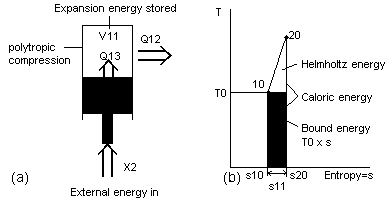Fig 19 The helmholtz ratio method vs entropy in gas compression process     In present textbooks the amount of external energy that could be obtained from a flow of caloric energy is always calculated using a term called entropy. Entropy often is confusing to people learning energy . This is because entropy is often implied as being a type of energy. It is not. Previously the unit of energy called bound energy was mentioned. Entropy...is a number that can be multiplied by the ambient temperature of Reservoir T0 to obtain this same bound energy. Entropy is used in processes where the properties of matter are not uniform with temperature and pressure and so cannot be easily calculated with formulas. Because of this, tables are used that list entropy at these different conditions. Instead of estimating the bound energy from a graph such as Fig 18 or calculating it from Eq (8), the values of entropy corresponding to the conditions at T2 and T1 are looked up. The difference of these two figures represents the changing entropy during the process. The change in the bound energy can then be calculated. Only one table is required with entropy even though calculations are done at different ambient temperatures. It is possible to use a property such as entropy because the Reservoir T0 does not change in temperature during the conversion.     Presently entropy is generally used in a subtractive-additive equation such as shown in Eq (13). This is the standard equation for calculating the helmholtz energy. Because such an equation does not calculate the helmholtz ratio first, it is less useful. This can be illustrated with the help of the diagrams of Fig 19. In Fig 19a there is an inefficient polytropic gas compression shown. In a polytropic compression process part of the thermal energy flows out of the system. In an inefficient process part of the expansion energy is converted into thermal energy. If such a process is calculated with entropy, it will be found that at the end of the compression process the helmholtz energy of the two streams of caloric energy would be combined into one amount. This is illustrated in Fig 19b. Only one amount of caloric energy, helmholtz energy and bound energy are possible for an individual process. The helmholtz ratio however can be multiplied by the caloric energy of each stream of energy by using a variation of Eq (11). (Eq 13) AE=QE-(T0·S) where S is the possible change in entropy of two states of the system such as s11 of Fig 19     The numbers used in entropy have little meaning by themselves, while the helmholtz ratio can be a useful figure by itself. It is a ratio or a percentage. If the helmholtz ratio of a certain system is 0.50 or 50%, it can immediately be visualized that a maximum of 50% of the caloric energy could be converted to external energy. Because most calculations are generally done at a set ambient temperature of 15°C, the helmholtz energy does not need to be recalculated very often for each process.     It is often said that the entropy of the universe is increasing, but it is less confusing to say that the clausius energy in the universe is decreasing.     In present textbooks, gibbs energy is often used, while in this booklet it is not. Gibbs energy is a mixture of helmholtz energy, which is internal energy and expansion energy, which is external energy. Because gibbs energy is such a mixture it cannot be used as a pure ratio in the same way that helmholtz energy can be. Its use is therefore more limited when doing calculations.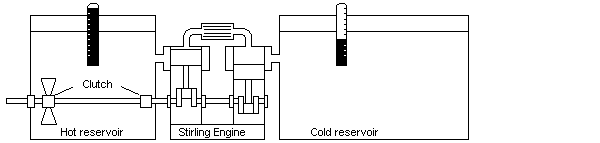Fig 20 Model demonstrating energy conversion and the carnot ratio     It is often said that the multi-directional motion of the molecules is more disordered than the directional energy of external energy and this is the reason it cannot be entirely converted into external energy. The concept of entropy, which was discussed in detail in Chapter 22, is largely understood in terms of disorder. The gas expansion examined in Chapter 10 is multi-directional energy however and it is considered to be a form of external energy. This is proof that multidirectional energy can be "straightened" entirely into external energy without losses in some cases. So it is difficult to relate order or disorder with a systems ability to be converted entirely in external energy. Because of this it is better to relate to the concept of reversible and irreversible processes. A reversible process...is a process which once having taken place, can be reversed back to the original conditions by some sort of process and in doing so leave no change in either the system or the surroundings. An irreversible process...is a process which once having taken place, cannot be reversed back to the original conditions by some sort of process and in doing so leave no change in either the system or the surroundings. It is really changes in the total order in the universe that are important. External energy can be perfectly stored in many systems that are disorderly, but if there is no change in the degree of disorder in the universe, then the system is reversible. This is true as well when working with entropy, the total entropy of a system is usually unimportant, it is rather the change in entropy that is important.     To understand reversibility better, the theoretical apparatus as shown in Fig 19 will be used. This is similar to the one in Fig 17 except that a Stirling engine is used to represent the U-X converter. While this apparatus only demonstrates transfers of thermal energy, the same reasoning applies equally to all other types of internal energy and any type of conversion mechanism. The basis for the apparatus are two reservoirs of water which store thermal energy. Again it is assumed that Reservoir T0 is infinitely larger than Reservoir T. A Stirling engine is placed between the tanks. This engine like the Carnot engine can theoretically convert all the helmholtz energy available in the caloric energy into external energy. This engine can also be used in reverse as a heat pump and can convert a quantity of external energy into caloric energy. These experiments are considered to be theoretically perfect.     In Experiment 1 we start with both reservoirs at equilibrium, meaning that they are at the same temperature. We transfer 10 units of external energy through the shaft to drive the Stirling engine as a heat pump. We find that the temperature of the ambient reservoir did not change appreciably but the Reservoir T became hotter. We have developed an amount of caloric energy between the two reservoirs. A possible amount is 50 units of caloric energy. If we convert the Stirling heat pump into a Stirling engine, we will find that this 50 units of caloric energy can be converted back into exactly 10 units of external energy, the same as we put in originally.     In Experiment 2 we start with both reservoirs at the same temperature. We transfer 10 units of external energy to turn the impeller in Reservoir T. This external energy is converted into caloric energy and the temperature of Reservoir T becomes hotter. The 10 units of external energy were converted into 10 units of caloric energy because they were made frictionally in a single reservoir system. If we took these 10 units of caloric energy we could obtain perhaps only 2 units of external energy back using the Stirling engine. We retrieve less external energy than we originally put in.     We can see in Experiment 1 that external energy can be reversibly stored in a system of thermal energy. There needs to be no loss of external energy. If however the external energy is converted in an irreversible manner as in Experiment 2, then there is a loss of external energy. What creates this irreversibility? It is a change in possible temperature that results in irreversibility. Instead of the external energy being used to turn the impeller in the large reservoir of water, we could have arranged to use a much smaller one. There is theoretically no limit to the temperature that could be created by this friction if an infinitely small reservoir was used. If all of the external energy was converted at an infinitely high temperature and flowed into the Stirling engine system as above and could be converted without melting the engine, we would be able to extract almost all of the external energy that we originally transferred into the system. The system would be almost completely reversible. So it is not friction that creates the irreversibility, it is the loss of temperature from the maximum that could be achieved that does it. According to the Energy Conversion Law...in any particular process of converting external energy to internal energy or internal energy to external energy, any temperature that results that is less than the maximum possible for that process is a loss of external energy.     It can be seen that internal energy and external energy are almost like two completely different entities and yet they still comply with the First Law of Energy. In one case they are treated as equals and in the other they are not.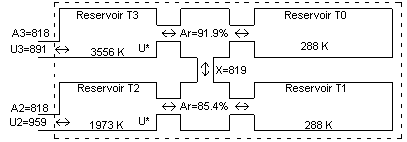Fig 21 Low amount of caloric energy made into high amount of caloric energy in an energy transformer     In an electrical transformer, high voltage low current power can be efficiently converted into low voltage high current power. Internal energy of a high temperature but low caloric energy content can also be converted into low temperature but high caloric energy content. Such a transformation is simplistically modeled in Fig 21 by connecting two system diagrams from Fig 17 together. In this diagram it can be shown how one form of internal energy with a high temperature such as methane fuel could be converted into another type of internal energy of a lower temperature but higher internal energy. Methane of 891 kJ enters Reservoir T3. This chemical energy has a high mean chemical temperature of about 3,500°K. X-energy of 819 kJ is extracted and transferred to another caloric energy system where 10,254 kJ of internal energy is created at 313°K thermal temperature. About 11.6 times as much caloric energy was created than was originally put in. The internal energy that is transformed can be thermal energy, latent energy, chemical energy etc. In the plural energy system different types of energy can be created but the total einstein energy of course is the same.     This type of internal energy transformer can actually be built in real life by using a fuel cell coupled to a heat pump. Theoretically the fuel cell can convert all of the 819 kJ helmholtz energy in the fuel to 819 kJ of electricity. The electricity created can be used to turn an electric motor, which converts the electricity to 819 kJ of mechanical energy, and drives a heat pump. The heat pump takes 9,435 kJ of thermal energy from Reservoir T1 and adds the 819 kJ of mechanical energy to create a total of 10,254 kJ of caloric energy. Notice that the 819 kJ of helmholtz energy in the fuel is the same as the 819 kJ of helmholtz energy in the thermal energy produced but the amount of caloric energy was increased. In actual practice the total caloric energy produced would be less due to inefficiencies in the conversion process.

 25. EFFICIENCY TERMS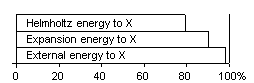Fig 22 Efficiency of converting some forms of energy to X-energy in large gas turbines     Presently it is said that certain steam engines are 30% efficiency in converting thermal energy into external energy. In the system proposed in this booklet however efficiency when used by itself is a word that is used only when converting similar types of energy. Because the First Law of Energy applies to all processes, they will all be 100% efficient in converting einstein energy to einstein energy. In a sense, internal energy and external energy are like two completely different energy entities. Because a larger amount of caloric energy is turned into a smaller amount of external energy or vis versa in a perfectly reversible process, this shouldn't be called an efficiency either. In the present system a refrigerator can have an efficiency higher than 100%, which does not sound right in this case and so a term of coefficient of performance is used.     Efficiency generally should be a term reserved for calculating what the conversion efficiency is between forms of energy that can be entirely converted into external energy. These forms are external, kinetic, potential, electrical, mechanical, expansion, helmholtz, gibbs, free, and clausius energy. For example the expression A-X efficiency can be used to represent the percentage of helmholtz energy that can be converted to external energy. In a large combined cycle gas turbine as shown in Fig 27, the after combustion A-M efficiency is up to 80%. This means that of the helmholtz energy left in the combustion chamber after the combustion process, 80% can be converted into mechanical energy. The expansion energy of the compressed gases can be converted at up to 90% V-M efficiency in the turbine. The mechanical energy produced by the shaft of the turbine can be converted to electricity at up to 99% M-W efficiency. If the efficiency of a process is based on internal energy, the efficiency at least should be clearly stated using symbols as suggested. This way it can clearly be seen which types of energy the efficiency is based on.     One of the big reasons for using the plural energy system is that common energy terms along with their abbreviations such as X, V, A can be used in these efficiency expressions. It is now possible to talk about efficiency for specific forms of energy rather than one broad one. 26. IS PERPETUAL MOTION POSSIBLE?     The world of internal energy is a world of constant "perpetual motion". In modern science though, perpetual motion does not refer to the goings on in the internal world, it is the ability to achieve endless external energy. The question that has been asked so often over the centuries is whether perpetual motion is possible. Basically two forms of perpetual motion have been visualized. Perpetual motion of the 1st kind...represents creating external energy without any other removal of einstein energy from the universe. Perpetual motion of the 2nd kind...represents the ability to convert external energy from internal energy that contains no helmholtz energy. There is presently little evidence that perpetual motion of the 1st kind is possible. Perpetual motion of the 2nd kind is also thought to be impossible by modern scientists, however there is no absolute scientific principle involved in this case. It is known that presently all processes seem to be limited by the equilibrium laws, but there is no way of telling whether there might be some undiscovered twist of nature where this does not apply.     Thousands of men have searched for ways to convert the seemingly endless thermal energy contained in the environment around us into useful external energy. It appears James Joule who introduced to science the concept of conservation of energy, believed that this would be possible. James Maxwell, the famous 1880s scientist, joked about a very tiny demon, which might be pressed into service to separate quickly moving molecules from slower molecules by operating a trap door between two chambers as shown in Fig 23. A fact taught in high school chemistry is that the temperature of a substance is only a measure of the average energy of the molecules. Figure 23 shows a bell curve of the range of energies that a typical gas would have. At any instant of time some molecules contain more and some less energy. These two reservoirs of energy produced by the demon could then be used to operate an engine to produce external energy.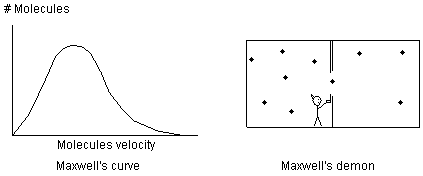Fig 23 Maxwell joked about a demon that would sort hot and cold molecules     A more modern perpetual motion concept is visualized by separating light with prisms or filters. Why try and separate hot and cold molecules when you can separate hot and cold photons that are emitted by the hot and cold molecules? Instead of trying to separate a heavy molecule with only a tiny bit of velocity energy contained it its motion, the photon represents raw, pure velocity energy. It is known that white light can be separated with a prism into photons of different energies or temperature. It is also known that at room temperature many substances are emitting photons of various energies of infrared light. Would it not be possible to separate these photons into different colors with a prism or a filter as shown in Fig 24 and then use the high and low temperature light to operate a heat engine? Apparently not. So far all the mechanisms that have been dreamed up all comply with the rule of equilibrium  and don't result in any production of external energy.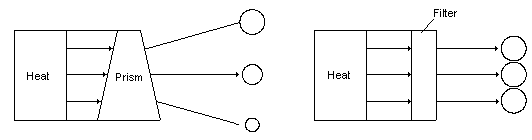Fig 24 Using prisms and filters as Maxwell's demon     Some have suggested that a heat pump or refrigerator could be used to create more energy than was put in. A heat pump or refrigerator cannot create any more helmholtz energy in an amount of caloric energy than the external energy put in. This helmholtz energy is the external energy stored in the heat.     It is a well known fact that at night, a small amount of thermal energy can be radiated out from the earth back into space. If for example heat is collected during the day, then cold is created during the night, it would be possible to run a heat engine and create external energy. It is true that a lot of different types of engines could be operated on the cycles of temperature that are created this way. This is not really a form of perpetual motion. Perpetual motion is based on extraction of external energy from a source of internal energy of constant temperature. The average temperature of outer space is considered to be about 3°K. Of course if it were possible to tap into this cold reservoir, heat engines could be operated very efficiently. Because any radiating surface is immersed in photons of ambient temperature, it is not possible to make use of the extreme cold of space on earth.     In the 1800's scientists argued whether gases in a column would stratify due to gravity and become hotter at the bottom than the top. Maxwell and Boltzmann said it would not, but Loschmidt a prominent scientist of his time  argued that the world would have a never-ending supply of energy this way. More modern experiments have been done using centrifugal force. There have been no working models to date.     Can chemical substances or biological living organisms not be found that can store only the energy contained in the photons or molecules of larger energy as shown in the bell curve of Fig 23? Could they not operate as storage devices with one way valves? Apparently not. They comply with the rules the same way as other processes.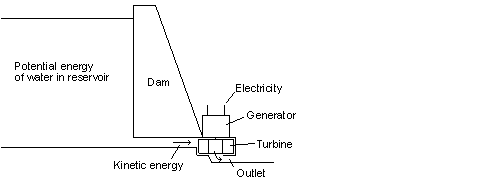Fig 25 The water stored behind the dam is a form of external energy     In the hydro electric dam, the water stored at a higher elevation is a source of potential energy. It is converted to kinetic energy in the turbines and then to electrical energy. These are all forms of external energy. As with the pendulum example in Fig 9, different forms of external energy can theoretically be entirely converted to other forms of external energy. While not every conversion is as simple as in the case of the pendulum, conversion is easier than when converting internal energy to external energy. Generally more than 90% of the potential energy of the water can be converted into electrical energy, which means that the P-W efficiency is 90%. It is also very easy to start and stop any one of the turbines and there is little loss in doing so. Hydro electric dams are perfect for supplying peaking power during periods of the day when more electric power is used than average. The potential energy that is not converted to electric energy is converted into thermal energy. This is done at such low temperatures that almost no helmholtz energy is left in the thermal energy.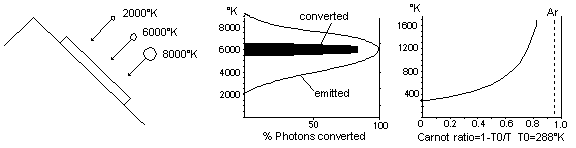Fig 26 Solar cells presently cannot utilize all the energy of sunlight     The light from the sun has an average temperature of about 6,300°K. The carnot ratio diagram of Fig 26 shows that the helmholtz ratio of sunlight is about 95%. This means that theoretically it should be possible to convert 95% of the radiant energy to electricity. In actual practice, present day common solar cells only convert about 11% of the radiant energy into electricity at most. This means that 89% of the remaining sunlight is converted into thermal energy. Solar cells are actually sheets of semiconductor material. When the sunlight strikes the semiconductor an electrical potential is created by dislodging electrons from the impact of the photons. Sunlight however is made of photons that contain different amounts of photon energy and different wavicle-frequency. The semiconductor cannot easily be tuned to receive all types efficiently. This means that some photons will not be converted at all because they have too little photon energy and some photons will only have a part of their energy converted to electricity because they have too much photon energy as shown in Fig 26. Does the temperature of the photons change on cloudy days? Only slightly. When a magnifying glass is used to concentrate light on a small area a hole can be burned through the paper. The temperature of the bright spot of light is the same as the light before the magnifying glass but because the light was spread out so much, the heat generated was dissipated too quickly for its temperature to rise. The brightness of the light changed, but the average photon energy of the photons did not change.     Scientists in laboratories are working on solar cells that have multiple layers of different semiconductors. Each layer is tuned to photons with a specific photon energy. Another type of solar cell first separates the light into different colors and then each color is converted in a different type of semiconductor. This results in a higher efficiency. Some types of solar cells use lenses or parabolic concentrators, which convert the radiant energy into thermal energy. Such a lens increases the brightness of the light. It is easy to convert photons of various photon energy entirely into thermal energy. A vapor cycle engine is used to convert this thermal energy into electricity. This would seem less efficient but due to the present low efficiency of semiconductor solar cells, this process actually results in an efficiency of nearly 30% in larger installations. One of the advantages with the system is that during the night or on cloudy days fuel can be burned in a separate boiler to add energy to the system. With the right solar concentrator and better engines, A-W efficiencies over 50% are possible. It may one day be possible to reach nearly 80% A-W efficiency with some type of device.     It seems odd that such a high temperature source of energy as the sun would only result in such low efficiencies in a heat engine. For example a modern gas turbine combined cycle has up to 57% A-W efficiency and uses a much lower temperature of combustion than 6300°K. The dilemma with solar energy is that a "window" is required to let the sunlight into the process. This same window also allows radiant energy to escape back out. One-way coatings generally only work to keep the low temperature rays from escaping but not the high temperature ones. Another reason is that indirect heating of the powerplant is required and this results in lower peak temperatures than by using internal combustion powerplants.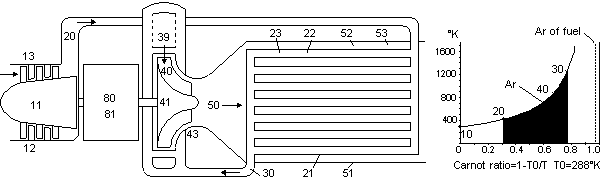Fig 27 Much of the temperature of the fuel in a heat engine is lost in the combustion chamber     Chemical energy or fuel represents a source of energy that can easily be stored, transported or piped to where it is needed. Fuel represents the most energy that can be obtained from a kilogram of substance next to using nuclear energy. Unfortunately fuel is internal energy. This type of energy is very hard to convert to external energy, which is the type that is needed for producing electricity or propelling vehicles. Engines such as steam engines and diesel engines must first convert the temperature of the chemical energy to thermal energy and then expand the hot gases produced to create external energy. A typical fuel such as jet fuel has a maximum chemical temperature of up to 20,000°K. This can represent a helmholtz ratio of up to 99%. There are two reasons why such a high temperature cannot be used in an engine. First, the products of the combustion process would dissociate at such a high temperature and absorb the energy, thereby lowering the final temperature produced. This is called the equilibrium temperature. Secondly no present day engine can properly utilize even the equilibrium temperature without melting or oxidizing the engine parts. Cooling the combustion gases before expansion results in a lower helmholtz ratio of the combustion gases, which means that less external energy could be extracted from the combustion gases in the gas turbine.     In the 1800s steam engines were widely used to generate electricity and power locomotives and ships. Liquid water was converted into steam by heating, which then pushed pistons or turned turbines. Today for larger engines the gas turbine is favored. This type of engine uses air as the expansion medium. In a gas turbine, air is compressed in the compressor, then fuel is added and burned, and lastly the hot gases are expanded in the turbine. Jet engines used in jet airplanes use a type of gas turbine that uses an expansion turbine that is only large enough to compress the incoming air. The thrust to drive the plane forward is created by the quickly moving hot combustion gases. Modern jet engines use a much larger turbine, which then drives a large ducted fan in the front of the engine. Most of the thrust is created as a result of the fan. This same type of gas turbine is used to generate electricity in large electricity generating stations.     Aircraft gas turbines operate with a high pressure cycle. This means that they compress the air to a high pressure before combustion. There are two reasons for running at a high pressure ratio. If we look at our carnot ratio chart for the low pressure gas turbine in Fig 27 it can be seen that the temperature at the end of the compression is low at point 20. If thermal energy was added to the combustion chamber at this point, most of the temperature of the fuel would be lost at the start of combustion. The helmholtz ratio of the fuel is 90% and the helmholtz energy retained at point 20 is only 31%. As well if the gases in a low pressure ratio turbine are expanded, only a small amount of thermal energy could be converted into external energy. In the high pressure gas turbine the temperature at the end of compression is much higher and less helmholtz energy is lost. The hot gases are expanded to a greater extent and more external energy is extracted.     A gas turbine can be even more efficient by operating at a low pressure ratio as shown in Fig 27 and using a heat exchanger. In the low pressure gas turbine much of the heat from the hot exhaust gases leaving the turbine can be recycled to the incoming colder compressed air by using a heat exchanger. This however is not the only reason for using a heat exchanger. The air coming into the combustion chamber from the heat exchanger can often be even hotter than in the high pressure cycle and so less helmholtz energy is lost.     It is common to talk about the efficiency of a heat engine in terms of the chemical energy that is burned. This is not really a fair comparison. As will seen in the next section on fuel cells, the helmholtz ratio of natural gas fuel is about 90%. This is the amount of external energy that is stored in the fuel. This means that theoretically 100 units of fuel or chemical energy could be produced with an input of only 90 units of external energy. It is better to compare the amount of external energy that can be obtained vs the amount of external energy stored in the fuel in the form of helmholtz energy, which is the A-X efficiency.     This may not seem to be very important when a fuel with a helmholtz ratio of 90-99%. Some chemical processes however operate at much lower helmholtz ratios, some as low as 10%. If we used a heat pump to create a chemical of this type, it appears that we have made more energy than we put in. If the fuel is rated by it's helmholtz energy, it will be much clearer that the clausius energy of the process did not increase.

 30. ELECTROCHEMICAL FUEL CELLS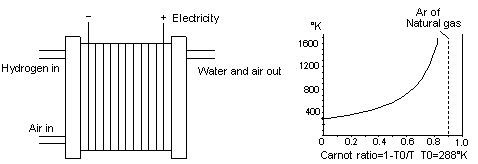Fig 29 A fuel cell can convert the temperature of the fuel directly into electricity     Heat engines are not available that can run hot enough to properly use the chemical temperature of fuels. A fuel cell creates electrical energy, which is a form of external energy, directly from the chemical temperature in fuels without an intermediate conversion into thermal energy. This is possible because the high temperature of the fuel energy was converted into electrical energy through the action of virtual photons as discussed in Chapter 15. In this way it can theoretically convert all the helmholtz energy of the fuel to electrical energy. As shown in Fig 29, natural gas fuel would have a helmholtz ratio of greater than 0.90 or 90%. This means that theoretically it would be possible to convert 90% of the fuel's internal energy into electricity. In actual practice there are a lot of frictional losses in the fuel cell. In a fuel cell, the helmholtz energy in the fuel is converted into ions, these are atoms with less or more the normal amount of electrons. Frictional losses due to movements of these ions, and of the electricity that is created, cause considerable amounts of thermal energy to be produced.     Low temperature fuel cells that operate at 80°C and use hydrogen as a fuel can have real A-X efficiencies of up to 60%. Higher temperature fuel cells that operate at 1000°K though can use hydrocarbon fuels and could perhaps reach 80% efficiency, but only by using the large amount of thermal energy produced in a separate heat engine. Because this thermal energy is created at a high temperature, about 77% of this thermal energy produced in the fuel cell through frictional losses remains as helmholtz energy.     While a fuel cell can theoretically convert the helmholtz energy of chemical energy directly into electricity, the dream of cold fusion is to similarly be able to convert the helmholtz energy of nuclear energy directly into electricity without having to turn the nuclear energy into thermal energy first. It is true however that present experiments with cold fusion have mostly involved only converting the nuclear energy into thermal energy. There have been claims of some cold fusion cells producing more thermal energy than the electricity put in. It is not known in many of these cases whether more helmholtz energy was actually produced. This would of course be a requirement for the device to be creating useful power. So far it appears that cold fusion is all still a dream.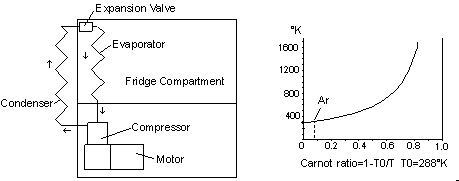Fig 30 A refrigerator creates more caloric energy than the external energy put in     In a refrigerator more caloric energy can be created than the external energy that was put in. In Chapter 23 we saw that by having two heat reservoirs, it is possible to create a potential flow of heat between them, which is called caloric energy. This caloric energy can be a flow from a hot reservoir to a cold ambient reservoir, or from a cold reservoir to a hotter ambient reservoir. If this caloric energy is created at a very small temperature difference, it does not take much external energy to create a larger amount of caloric energy. Theoretically it would be possible to create 10,000 joules or even more of caloric energy with only 1 joule of external energy. This does not mean that the 9,999 joules was obtained for free. According to the First Law of Energy all the einstein energy must add up. We are removing 9,999 joules of internal energy from one reservoir and pumping it into the other. If this reservoir is the earth there is an almost endless supply.     Theoretically then it is possible to create 13.65 joules of cold at 0 deg Celsius when the room temperature is 20 deg Celsius by adding 1.0 joule of external energy to a refrigeration device when the cold is produced at a constant temperature. In actual practice the processes do not occur at constant temperatures and a loss of efficiency results. The heat energy is disposed of by the condenser at the back of the refrigerator at variable temperatures much higher than room temperature and the evaporator draws heat energy inside the refrigerator at variable temperatures somewhat colder than the refrigerator compartment. Ar=AE/QE can be reorganized to QE=AE/Ar and assume that XE is turned entirely into AE QE=1.0 joule/0.07326 QE=13.65 joules     Is it possible to create a perpetual motion machine by creating 13.65 joules caloric energy with the 1.0 joule of external energy we put in, then using a heat engine to make more than 1.0 joule external energy back? No, the heat engine would be able to theoretically extract exactly 1.0 joule of external energy from the 13.65 joules of caloric energy that was created.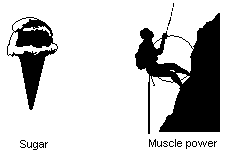Fig 31 Sugar is converted into muscle power at about 25% A-X efficiency     Human muscles don't convert the chemical energy in foods into heat first as is done in an engine such as a gas turbine. It is often thought that this must mean that muscles are very efficient in converting chemical energy into external energy. Tests have been done with cyclists, and it appears that the body only has a net 25% A-X efficiency in converting food into muscle energy.     Food is typically rated in Calories. Actually these are referred to as big calories or kilocalories in more exact scientific terminology. An average person eats around 3,000 kilocalories per day. Many food packages are also listing the food value in joules, something that is now preferred. The 3,000 kilocalories is the caloric energy of the food. The helmholtz energy of sugar is slightly less than this. If the climber shown in Fig 31 above weighed 150 lbs or 68 kg, then the maximum distance the climber could travel upwards with 3,000 kilocalories sugar energy at 25% A-X efficiency would be about 12,000 ft or 3,700 m. The same 3,000 kilocalories could be used to heat up about 60 liters of water 50°C, which would be just enough for a nice hot bath.     The body does not use sugar directly in the muscles. Through enzymes, the body is able to chemically change one complex molecule to another without losing too much helmholtz energy. The enzymes are able to take the very high temperature energy of one molecule, and carefully transfer it into another molecule without creating too much thermal energy as a byproduct. As has already been pointed out, this is because the energy is transferred by means of virtual photons, which spill very little of their high chemical temperature to the surrounding environment.Fig 32 The early steam engine converted less than 5% of helmholtz energy in the fuel to external energy Timeline BC 1700 Babylonians employ windmills to pump water for irrigation 500 Greeks proposed matter was tiny indivisible particles in motion AD 1510 A horizontal water wheel designed by Leonardo da Vinci 1633 Galileo is on trial for his belief that the earth rotates around the sun 1633 René Descartes proposes light travels in an aether which fills the entire universe 1666 Isaac Newton formulates the Laws of Gravity 1676 Romer estimates the Speed of Light based on celestial movements 1676 Isaac Newton formulates Particle theory of light 1690 Christian Huygens proposed that light was a wave phenomenon 1698 Thomas Savery develops steam pump 1705 Newcomen develops first steam engine 1769 James Watt invents higher efficiency steam engine 1790 Count Rumford does cannon boring experiment, says heat is a form of energy 1801 Thomas Young produces interference pattern with light, pronounces light a wave 1814 First steam locomotive by English inventor George Stephenson 1824 Carnot publishes book about Carnot's Law 1831 Michael Faraday and Joseph Henry independently discovered electromagnetic induction 1840 Joule discovers the relationship between work and heat 1849 Fizeau measures the speed of light reasonably accurately with shutter wheel and mirrors 1850 Clausius develops the concept of entropy 1851 Kelvin temperature scale developed by Lord Kelvin 1887 Michelson-Morley experiment did not detect different light speed in perpendicular light beams 1897 Electron was discovered by Joseph Thomson 1900 Planck proposed the quantum theory of radiation 1904 Lorentz proposes that objects change in dimension as they move through the ether 1905 Einstein's Special Theory of Relativity suggests light and matter are composed of the same energy 1911 Proton discovered 1925 Michelson-Gale experiment showed different light speed in counter-rotating light beams 1932 Neutron discovered 1945 First Atomic Bomb is exploded 1955 Miller's ether drift experiment concluded to be the result of experimental error 1962 Quarks, the building blocks of protons and neutrons theorized and soon measured 1989 Cold fusion thought to be occurring in experiments by Pons and Fleischmann 34. SYMBOLS MA = absolute mass M0 = rest mass MR =relativistic mass MV = velocity mass EA = absolute energy E0 = rest energy ER = relativistic energy EV = velocity energy AE = helmholtz energy BE = bound energy CE = chemical energy DE = cogen energy EE = einstein energy FE = free energy GE = gibbs energy HE = heat energy IE = nucleus energy KE = kinetic energy LE = latent energy ME = mechanical energy NE = nuclear energy PE = potential energy QE = caloric energy RE = radiant energy SE = solution energy TE = thermal energy UE = internal energy VE = expansion energy WE = electrical energy XE = external energy YE = clausius energy ZE = electron energy Gr = gibbs ratio Ar = helmholtz ratio Cr = carnot ratio J = joules=1 Newton-meter of energy T = temperature °K or °C T0 = ambient temperature, °K, represents infinitely large heat sink T = absolute temperature, °K of second reservoir of energy S = entropy kJ/kg-°K 10,11,20,30 = points on process or processes